# Open CASCADE Technology: Modeling Algorithms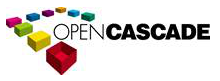Open CASCADE Technology  7.2.0
Modeling Algorithms

# Introduction

This manual explains how to use the Modeling Algorithms. It provides basic documentation on modeling algorithms. For advanced information on Modeling Algorithms, see our E-learning & Training offerings.

The Modeling Algorithms module brings together a wide range of topological algorithms used in modeling. Along with these tools, you will find the geometric algorithms, which they call.

# Geometric Tools

Open CASCADE Technology geometric tools provide algorithms to:

• Calculate the intersection of two 2D curves, surfaces, or a 3D curve and a surface;
• Project points onto 2D and 3D curves, points onto surfaces, and 3D curves onto surfaces;
• Construct lines and circles from constraints;
• Construct curves and surfaces from constraints;
• Construct curves and surfaces by interpolation.

## Intersections

The Intersections component is used to compute intersections between 2D or 3D geometrical objects:

• the intersections between two 2D curves;
• the self-intersections of a 2D curve;
• the intersection between a 3D curve and a surface;
• the intersection between two surfaces.

The Geom2dAPI_InterCurveCurve class allows the evaluation of the intersection points (gp_Pnt2d) between two geometric curves (Geom2d_Curve) and the evaluation of the points of self-intersection of a curve.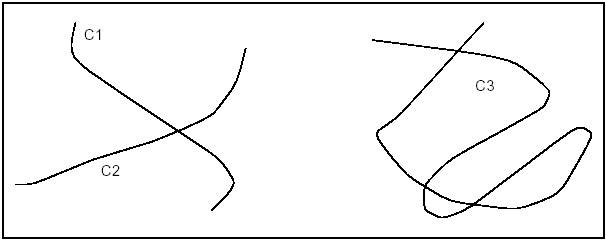Intersection and self-intersection of curves

In both cases, the algorithm requires a value for the tolerance (Standard_Real) for the confusion between two points. The default tolerance value used in all constructors is 1.0e-6.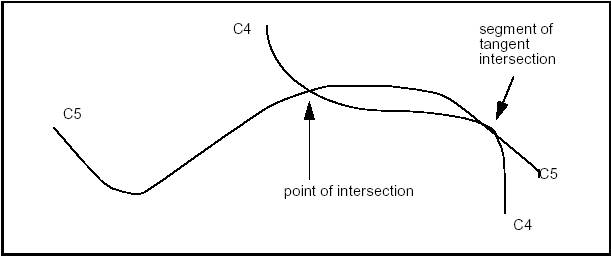Intersection and tangent intersection

The algorithm returns a point in the case of an intersection and a segment in the case of tangent intersection.

### Intersection of two curves

Geom2dAPI_InterCurveCurve class may be instantiated for intersection of curves C1 and C2.

Geom2dAPI_InterCurveCurve Intersector(C1,C2,tolerance);

or for self-intersection of curve C3.

Geom2dAPI_InterCurveCurve Intersector(C3,tolerance);
Standard_Integer N = Intersector.NbPoints();

Calls the number of intersection points

To select the desired intersection point, pass an integer index value in argument.

gp_Pnt2d P = Intersector.Point(Index);

To call the number of intersection segments, use

Standard_Integer M = Intersector.NbSegments();

To select the desired intersection segment pass integer index values in argument.

Handle(Geom2d_Curve) Seg1, Seg2;
Intersector.Segment(Index,Seg1,Seg2);
// if intersection of 2 curves
Intersector.Segment(Index,Seg1);
// if self-intersection of a curve

If you need access to a wider range of functionalities the following method will return the algorithmic object for the calculation of intersections:

Geom2dInt_GInter& TheIntersector = Intersector.Intersector();

### Intersection of Curves and Surfaces

The GeomAPI_IntCS class is used to compute the intersection points between a curve and a surface.

This class is instantiated as follows:

GeomAPI_IntCS Intersector(C, S);

To call the number of intersection points, use:

Standard_Integer nb = Intersector.NbPoints();
gp_Pnt& P = Intersector.Point(Index);

Where Index is an integer between 1 and nb, calls the intersection points.

### Intersection of two Surfaces

The GeomAPI_IntSS class is used to compute the intersection of two surfaces from Geom_Surface with respect to a given tolerance.

This class is instantiated as follows:

GeomAPI_IntSS Intersector(S1, S2, Tolerance);

Once the GeomAPI_IntSS object has been created, it can be interpreted.

Standard_Integer nb = Intersector. NbLines();

Calls the number of intersection curves.

Handle(Geom_Curve) C = Intersector.Line(Index)

Where Index is an integer between 1 and nb, calls the intersection curves.

## Interpolations

The Interpolation Laws component provides definitions of functions: y=f(x) .

In particular, it provides definitions of:

• a linear function,
• an S function, and
• an interpolation function for a range of values.

Such functions can be used to define, for example, the evolution law of a fillet along the edge of a shape.

The validity of the function built is never checked: the Law package does not know for what application or to what end the function will be used. In particular, if the function is used as the evolution law of a fillet, it is important that the function is always positive. The user must check this.

### Geom2dAPI_Interpolate

This class is used to interpolate a BSplineCurve passing through an array of points. If tangency is not requested at the point of interpolation, continuity will be C2. If tangency is requested at the point, continuity will be C1. If Periodicity is requested, the curve will be closed and the junction will be the first point given. The curve will then have a continuity of C1 only. This class may be instantiated as follows:

Geom2dAPI_Interpolate
(const Handle_TColgp_HArray1OfPnt2d& Points,
const Standard_Boolean PeriodicFlag,
const Standard_Real Tolerance);
Geom2dAPI_Interpolate Interp(Points, Standard_False,
Precision::Confusion());

It is possible to call the BSpline curve from the object defined above it.

Handle(Geom2d_BSplineCurve) C = Interp.Curve();

Note that the Handle(Geom2d_BSplineCurve) operator has been redefined by the method Curve(). Consequently, it is unnecessary to pass via the construction of an intermediate object of the Geom2dAPI_Interpolate type and the following syntax is correct.

Handle(Geom2d_BSplineCurve) C =
Geom2dAPI_Interpolate(Points,
Standard_False,
Precision::Confusion());

### GeomAPI_Interpolate

This class may be instantiated as follows:

GeomAPI_Interpolate
(const Handle_TColgp_HArray1OfPnt& Points,
const Standard_Boolean PeriodicFlag,
const Standard_Real Tolerance);
GeomAPI_Interpolate Interp(Points, Standard_False,
Precision::Confusion());

It is possible to call the BSpline curve from the object defined above it.

Handle(Geom_BSplineCurve) C = Interp.Curve();

Note that the Handle(Geom_BSplineCurve) operator has been redefined by the method Curve(). Thus, it is unnecessary to pass via the construction of an intermediate object of the GeomAPI_Interpolate type and the following syntax is correct.

Handle(Geom_BSplineCurve) C = GeomAPI_Interpolate(Points, Standard_False, 1.0e-7);

Boundary conditions may be imposed with the method Load.

GeomAPI_Interpolate AnInterpolator
(Points, Standard_False, 1.0e-5);

## Lines and Circles from Constraints

### Types of constraints

The algorithms for construction of 2D circles or lines can be described with numeric or geometric constraints in relation to other curves.

These constraints can impose the following :

• the radius of a circle,
• the angle that a straight line makes with another straight line,
• the tangency of a straight line or circle in relation to a curve,
• the passage of a straight line or circle through a point,
• the circle with center in a point or curve.

For example, these algorithms enable to easily construct a circle of a given radius, centered on a straight line and tangential to another circle.

The implemented algorithms are more complex than those provided by the Direct Constructions component for building 2D circles or lines.

The expression of a tangency problem generally leads to several results, according to the relative positions of the solution and the circles or straight lines in relation to which the tangency constraints are expressed. For example, consider the following case of a circle of a given radius (a small one) which is tangential to two secant circles C1 and C2: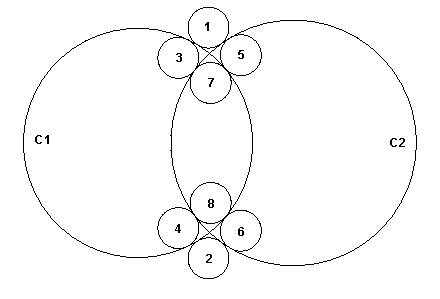Example of a Tangency Constraint

This diagram clearly shows that there are 8 possible solutions.

In order to limit the number of solutions, we can try to express the relative position of the required solution in relation to the circles to which it is tangential. For example, if we specify that the solution is inside the circle C1 and outside the circle C2, only two solutions referenced 3 and 4 on the diagram respond to the problem posed.

These definitions are very easy to interpret on a circle, where it is easy to identify the interior and exterior sides. In fact, for any kind of curve the interior is defined as the left-hand side of the curve in relation to its orientation.

This technique of qualification of a solution, in relation to the curves to which it is tangential, can be used in all algorithms for constructing a circle or a straight line by geometric constraints. Four qualifiers are used:

• Enclosing – the solution(s) must enclose the argument;
• Enclosed – the solution(s) must be enclosed by the argument;
• Outside – the solution(s) and the argument must be external to one another;
• Unqualified – the relative position is not qualified, i.e. all solutions apply.

It is possible to create expressions using the qualifiers, for example:

Solver(GccEnt::Outside(C1),

This expression finds all circles of radius Rad, which are tangent to both circle C1 and C2, while C1 is outside and C2 is inside.

### Available types of lines and circles

The following analytic algorithms using value-handled entities for creation of 2D lines or circles with geometric constraints are available:

• circle tangent to three elements (lines, circles, curves, points),
• circle tangent to two elements and having a radius,
• circle tangent to two elements and centered on a third element,
• circle tangent to two elements and centered on a point,
• circle tangent to one element and centered on a second,
• bisector of two points,
• bisector of two lines,
• bisector of two circles,
• bisector of a line and a point,
• bisector of a circle and a point,
• bisector of a line and a circle,
• line tangent to two elements (points, circles, curves),
• line tangent to one element and parallel to a line,
• line tangent to one element and perpendicular to a line,
• line tangent to one element and forming angle with a line.

#### Exterior/Interior

It is not hard to define the interior and exterior of a circle. As is shown in the following diagram, the exterior is indicated by the sense of the binormal, that is to say the right side according to the sense of traversing the circle. The left side is therefore the interior (or "material").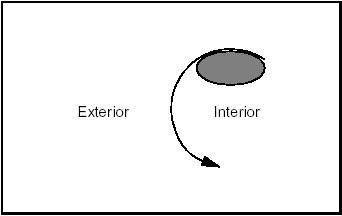Exterior/Interior of a Circle

By extension, the interior of a line or any open curve is defined as the left side according to the passing direction, as shown in the following diagram: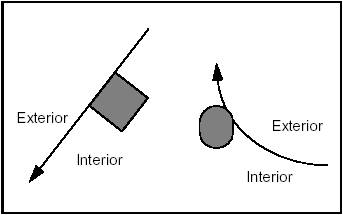Exterior/Interior of a Line and a Curve

#### Orientation of a Line

It is sometimes necessary to define in advance the sense of travel along a line to be created. This sense will be from first to second argument.

The following figure shows a line, which is first tangent to circle C1 which is interior to the line, and then passes through point P1.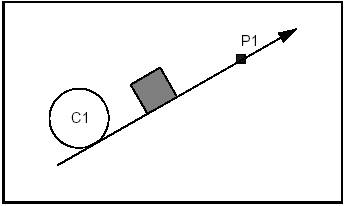An Oriented Line

#### Line tangent to two circles

The following four diagrams illustrate four cases of using qualifiers in the creation of a line. The fifth shows the solution if no qualifiers are given.

Example 1 Case 1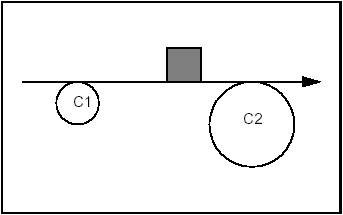Both circles outside

Constraints: Tangent and Exterior to C1. Tangent and Exterior to C2.

Syntax:

GccAna_Lin2d2Tan
Solver(GccEnt::Outside(C1),
GccEnt::Outside(C2),
Tolerance);

Example 1 Case 2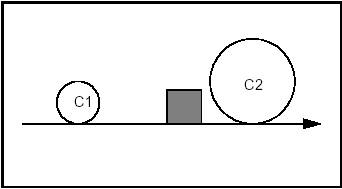Both circles enclosed

Constraints: Tangent and Including C1. Tangent and Including C2.

Syntax:

GccAna_Lin2d2Tan
Solver(GccEnt::Enclosing(C1),
GccEnt::Enclosing(C2),
Tolerance);

Example 1 Case 3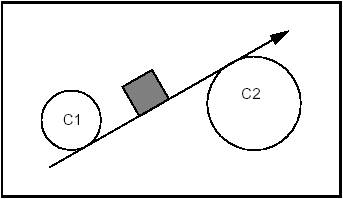C1 enclosed and C2 outside

Constraints: Tangent and Including C1. Tangent and Exterior to C2.

Syntax:

GccAna_Lin2d2Tan
Solver(GccEnt::Enclosing(C1),
GccEnt::Outside(C2),
Tolerance);

Example 1 Case 4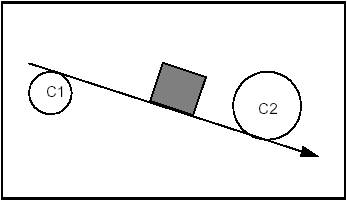C1 outside and C2 enclosed

Constraints: Tangent and Exterior to C1. Tangent and Including C2.

Syntax:

GccAna_Lin2d2Tan
Solver(GccEnt::Outside(C1),
GccEnt::Enclosing(C2),
Tolerance);

Example 1 Case 5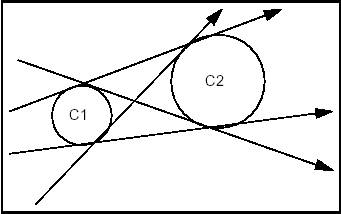Without qualifiers

Constraints: Tangent and Undefined with respect to C1. Tangent and Undefined with respect to C2.

Syntax:

GccAna_Lin2d2Tan
Solver(GccEnt::Unqualified(C1),
GccEnt::Unqualified(C2),
Tolerance);

#### Circle of given radius tangent to two circles

The following four diagrams show the four cases in using qualifiers in the creation of a circle.

Example 2 Case 1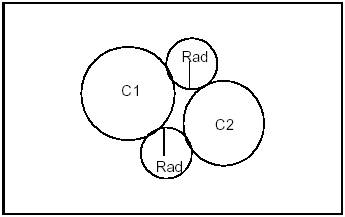Both solutions outside

Constraints: Tangent and Exterior to C1. Tangent and Exterior to C2.

Syntax:

Solver(GccEnt::Outside(C1),

Example 2 Case 2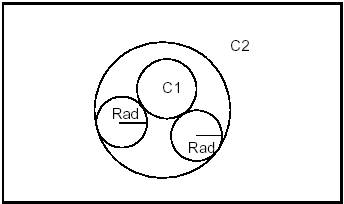C2 encompasses C1

Constraints: Tangent and Exterior to C1. Tangent and Included by C2.

Syntax:

Solver(GccEnt::Outside(C1),

Example 2 Case 3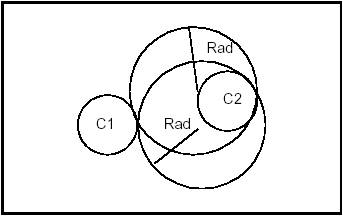Solutions enclose C2

Constraints: Tangent and Exterior to C1. Tangent and Including C2.

Syntax:

Solver(GccEnt::Outside(C1),

Example 2 Case 4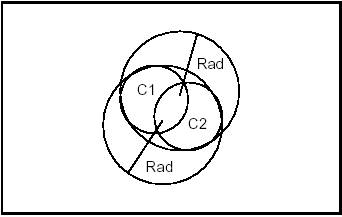Solutions enclose C1

Constraints: Tangent and Enclosing C1. Tangent and Enclosing C2.

Syntax:

Solver(GccEnt::Enclosing(C1),

Example 2 Case 5

The following syntax will give all the circles of radius Rad, which are tangent to C1 and C2 without discrimination of relative position:

GccEnt::Unqualified(C2),

### Types of algorithms

OCCT implements several categories of algorithms:

• Analytic algorithms, where solutions are obtained by the resolution of an equation, such algorithms are used when the geometries which are worked on (tangency arguments, position of the center, etc.) are points, lines or circles;
• Geometric algorithms, where the solution is generally obtained by calculating the intersection of parallel or bisecting curves built from geometric arguments;
• Iterative algorithms, where the solution is obtained by a process of iteration.

For each kind of geometric construction of a constrained line or circle, OCCT provides two types of access:

• algorithms from the package Geom2dGcc automatically select the algorithm best suited to the problem, both in the general case and in all types of specific cases; the used arguments are Geom2d objects, while the computed solutions are gp objects;
• algorithms from the package GccAna resolve the problem analytically, and can only be used when the geometries to be worked on are lines or circles; both the used arguments and the computed solutions are gp objects.

The provided algorithms compute all solutions, which correspond to the stated geometric problem, unless the solution is found by an iterative algorithm.

Iterative algorithms compute only one solution, closest to an initial position. They can be used in the following cases:

• to build a circle, when an argument is more complex than a line or a circle, and where the radius is not known or difficult to determine: this is the case for a circle tangential to three geometric elements, or tangential to two geometric elements and centered on a curve;
• to build a line, when a tangency argument is more complex than a line or a circle.

Qualified curves (for tangency arguments) are provided either by:

• the GccEnt package, for direct use by GccAna algorithms, or
• the Geom2dGcc package, for general use by Geom2dGcc algorithms.

The GccEnt and Geom2dGcc packages also provide simple functions for building qualified curves in a very efficient way.

The GccAna package also provides algorithms for constructing bisecting loci between circles, lines or points. Bisecting loci between two geometric objects are such that each of their points is at the same distance from the two geometric objects. They are typically curves, such as circles, lines or conics for GccAna algorithms. Each elementary solution is given as an elementary bisecting locus object (line, circle, ellipse, hyperbola, parabola), described by the GccInt package.

Note: Curves used by GccAna algorithms to define the geometric problem to be solved, are 2D lines or circles from the gp package: they are not explicitly parameterized. However, these lines or circles retain an implicit parameterization, corresponding to that which they induce on equivalent Geom2d objects. This induced parameterization is the one used when returning parameter values on such curves, for instance with the functions Tangency1, Tangency2, Tangency3, Intersection2 and CenterOn3 provided by construction algorithms from the GccAna or Geom2dGcc packages.

## Curves and Surfaces from Constraints

The Curves and Surfaces from Constraints component groups together high level functions used in 2D and 3D geometry for:

• creation of faired and minimal variation 2D curves
• construction of ruled surfaces
• construction of pipe surfaces
• filling of surfaces
• construction of plate surfaces
• extension of a 3D curve or surface beyond its original bounds.

OPEN CASCADE company also provides a product known as Surfaces from Scattered Points, which allows constructing surfaces from scattered points. This algorithm accepts or constructs an initial B-Spline surface and looks for its deformation (finite elements method) which would satisfy the constraints. Using optimized computation methods, this algorithm is able to construct a surface from more than 500 000 points.

SSP product is not supplied with Open CASCADE Technology, but can be purchased separately.

### Faired and Minimal Variation 2D Curves

Elastic beam curves have their origin in traditional methods of modeling applied in boat-building, where a long thin piece of wood, a lathe, was forced to pass between two sets of nails and in this way, take the form of a curve based on the two points, the directions of the forces applied at those points, and the properties of the wooden lathe itself.

Maintaining these constraints requires both longitudinal and transversal forces to be applied to the beam in order to compensate for its internal elasticity. The longitudinal forces can be a push or a pull and the beam may or may not be allowed to slide over these fixed points.

#### Batten Curves

The class FairCurve_Batten allows producing faired curves defined on the basis of one or more constraints on each of the two reference points. These include point, angle of tangency and curvature settings. The following constraint orders are available:

• 0 the curve must pass through a point
• 1 the curve must pass through a point and have a given tangent
• 2 the curve must pass through a point, have a given tangent and a given curvature.

Only 0 and 1 constraint orders are used. The function Curve returns the result as a 2D BSpline curve.

#### Minimal Variation Curves

The class FairCurve_MinimalVariation allows producing curves with minimal variation in curvature at each reference point. The following constraint orders are available:

• 0 the curve must pass through a point
• 1 the curve must pass through a point and have a given tangent
• 2 the curve must pass through a point, have a given tangent and a given curvature.

Constraint orders of 0, 1 and 2 can be used. The algorithm minimizes tension, sagging and jerk energy.

The function Curve returns the result as a 2D BSpline curve.

If you want to give a specific length to a batten curve, use:

b.SetSlidingFactor(L / b.SlidingOfReference())

where b is the name of the batten curve object

Free sliding is generally more aesthetically pleasing than constrained sliding. However, the computation can fail with values such as angles greater than p/2 because in this case the length is theoretically infinite.

In other cases, when sliding is imposed and the sliding factor is too large, the batten can collapse.

The constructor parameters, Tolerance and NbIterations, control how precise the computation is, and how long it will take.

### Ruled Surfaces

A ruled surface is built by ruling a line along the length of two curves.

#### Creation of Bezier surfaces

The class GeomFill_BezierCurves allows producing a Bezier surface from contiguous Bezier curves. Note that problems may occur with rational Bezier Curves.

#### Creation of BSpline surfaces

The class GeomFill_BSplineCurves allows producing a BSpline surface from contiguous BSpline curves. Note that problems may occur with rational BSplines.

### Pipe Surfaces

The class GeomFill_Pipe allows producing a pipe by sweeping a curve (the section) along another curve (the path). The result is a BSpline surface.

The following types of construction are available:

• pipes with a circular section of constant radius,
• pipes with a constant section,
• pipes with a section evolving between two given curves.

### Filling a contour

It is often convenient to create a surface from some curves, which will form the boundaries that define the new surface. This is done by the class GeomFill_ConstrainedFilling, which allows filling a contour defined by three or four curves as well as by tangency constraints. The resulting surface is a BSpline.

A case in point is the intersection of two fillets at a corner. If the radius of the fillet on one edge is different from that of the fillet on another, it becomes impossible to sew together all the edges of the resulting surfaces. This leaves a gap in the overall surface of the object which you are constructing.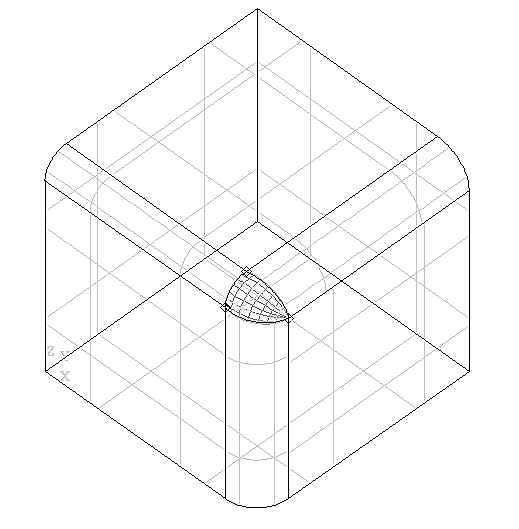Intersecting filleted edges with differing radiuses

These algorithms allow you to fill this gap from two, three or four curves. This can be done with or without constraints, and the resulting surface will be either a Bezier or a BSpline surface in one of a range of filling styles.

#### Creation of a Boundary

The class GeomFill_SimpleBound allows you defining a boundary for the surface to be constructed.

#### Creation of a Boundary with an adjoining surface

The class GeomFill_BoundWithSurf allows defining a boundary for the surface to be constructed. This boundary will already be joined to another surface.

#### Filling styles

The enumerations FillingStyle specify the styles used to build the surface. These include:

• Stretch – the style with the flattest patches
• Coons – a rounded style with less depth than Curved
• Curved – the style with the most rounded patches.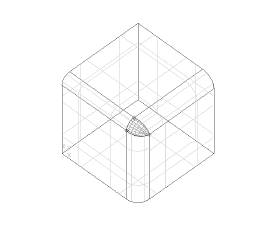Intersecting filleted edges with different radii leave a gap filled by a surface

### Plate surfaces

In CAD, it is often necessary to generate a surface which has no exact mathematical definition, but which is defined by respective constraints. These can be of a mathematical, a technical or an aesthetic order.

Essentially, a plate surface is constructed by deforming a surface so that it conforms to a given number of curve or point constraints. In the figure below, you can see four segments of the outline of the plane, and a point which have been used as the curve constraints and the point constraint respectively. The resulting surface can be converted into a BSpline surface by using the function MakeApprox .

The surface is built using a variational spline algorithm. It uses the principle of deformation of a thin plate by localised mechanical forces. If not already given in the input, an initial surface is calculated. This corresponds to the plate prior to deformation. Then, the algorithm is called to calculate the final surface. It looks for a solution satisfying constraints and minimizing energy input.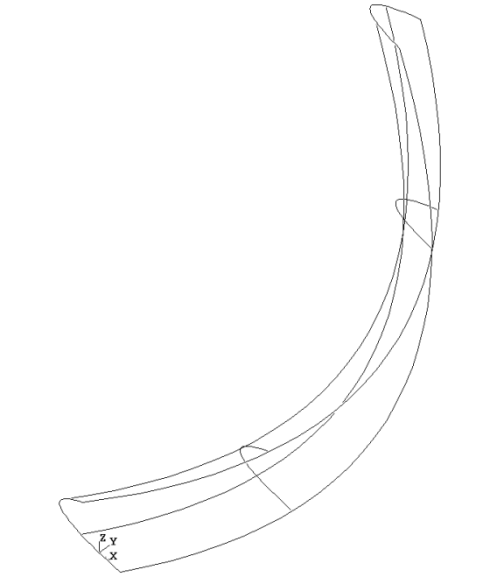Surface generated from two curves and a point

The package GeomPlate provides the following services for creating surfaces respecting curve and point constraints:

#### Definition of a Framework

The class BuildPlateSurface allows creating a framework to build surfaces according to curve and point constraints as well as tolerance settings. The result is returned with the function Surface.

Note that you do not have to specify an initial surface at the time of construction. It can be added later or, if none is loaded, a surface will be computed automatically.

#### Definition of a Curve Constraint

The class CurveConstraint allows defining curves as constraints to the surface, which you want to build.

#### Definition of a Point Constraint

The class PointConstraint allows defining points as constraints to the surface, which you want to build.

#### Applying Geom_Surface to Plate Surfaces

The class Surface allows describing the characteristics of plate surface objects returned by BuildPlateSurface::Surface using the methods of Geom_Surface

#### Approximating a Plate surface to a BSpline

The class MakeApprox allows converting a GeomPlate surface into a Geom_BSplineSurface.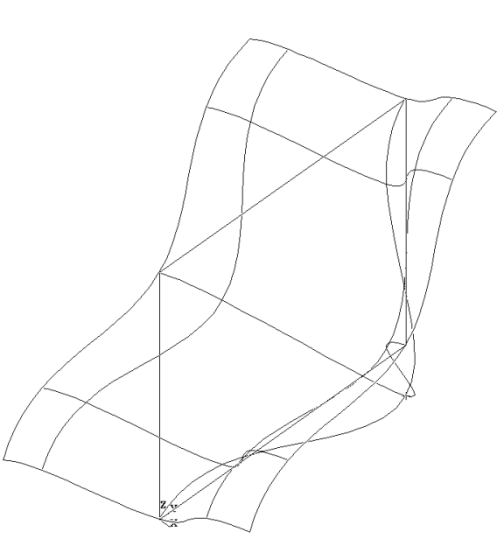Surface generated from four curves and a point

Let us create a Plate surface and approximate it from a polyline as a curve constraint and a point constraint

Standard_Integer NbCurFront=4,
NbPointConstraint=1;
gp_Pnt P1(0.,0.,0.);
gp_Pnt P2(0.,10.,0.);
gp_Pnt P3(0.,10.,10.);
gp_Pnt P4(0.,0.,10.);
gp_Pnt P5(5.,5.,5.);
BRepBuilderAPI_MakePolygon W;
// Initialize a BuildPlateSurface
GeomPlate_BuildPlateSurface BPSurf(3,15,2);
// Create the curve constraints
BRepTools_WireExplorer anExp;
for(anExp.Init(W); anExp.More(); anExp.Next())
{
TopoDS_Edge E = anExp.Current();
C-ChangeCurve().Initialize(E);
Handle(BRepFill_CurveConstraint) Cont= new
BRepFill_CurveConstraint(C,0);
}
// Point constraint
Handle(GeomPlate_PointConstraint) PCont= new
GeomPlate_PointConstraint(P5,0);
// Compute the Plate surface
BPSurf.Perform();
// Approximation of the Plate surface
Standard_Integer MaxSeg=9;
Standard_Integer MaxDegree=8;
Standard_Integer CritOrder=0;
Standard_Real dmax,Tol;
Handle(GeomPlate_Surface) PSurf = BPSurf.Surface();
dmax = Max(0.0001,10*BPSurf.G0Error());
Tol=0.0001;
GeomPlate_MakeApprox
Mapp(PSurf,Tol,MaxSeg,MaxDegree,dmax,CritOrder);
Handle (Geom_Surface) Surf (Mapp.Surface());
// create a face corresponding to the approximated Plate
Surface
Standard_Real Umin, Umax, Vmin, Vmax;
PSurf->Bounds( Umin, Umax, Vmin, Vmax);
BRepBuilderAPI_MakeFace MF(Surf,Umin, Umax, Vmin, Vmax);

## Projections

Projections provide for computing the following:

• the projections of a 2D point onto a 2D curve
• the projections of a 3D point onto a 3D curve or surface
• the projection of a 3D curve onto a surface.
• the planar curve transposition from the 3D to the 2D parametric space of an underlying plane and v. s.
• the positioning of a 2D gp object in the 3D geometric space.

### Projection of a 2D Point on a Curve

Geom2dAPI_ProjectPointOnCurve allows calculation of all normals projected from a point (gp_Pnt2d) onto a geometric curve (Geom2d_Curve). The calculation may be restricted to a given domain.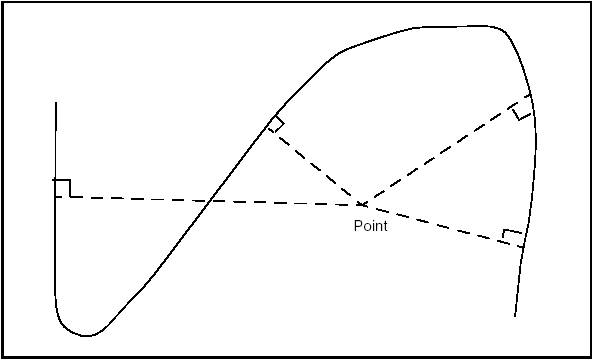Normals from a point to a curve

The curve does not have to be a Geom2d_TrimmedCurve. The algorithm will function with any class inheriting Geom2d_Curve.

The class Geom2dAPI_ProjectPointOnCurve may be instantiated as in the following example:

gp_Pnt2d P;
Handle(Geom2d_BezierCurve) C =
new Geom2d_BezierCurve(args);
Geom2dAPI_ProjectPointOnCurve Projector (P, C);

To restrict the search for normals to a given domain [U1,U2], use the following constructor:

Geom2dAPI_ProjectPointOnCurve Projector (P, C, U1, U2);

Having thus created the Geom2dAPI_ProjectPointOnCurve object, we can now interrogate it.

#### Calling the number of solution points

Standard_Integer NumSolutions = Projector.NbPoints();

#### Calling the location of a solution point

The solutions are indexed in a range from 1 to Projector.NbPoints(). The point, which corresponds to a given Index may be found:

gp_Pnt2d Pn = Projector.Point(Index);

#### Calling the parameter of a solution point

For a given point corresponding to a given Index:

Standard_Real U = Projector.Parameter(Index);

This can also be programmed as:

Standard_Real U;
Projector.Parameter(Index,U);

#### Calling the distance between the start and end points

We can find the distance between the initial point and a point, which corresponds to the given Index:

Standard_Real D = Projector.Distance(Index);

#### Calling the nearest solution point

This class offers a method to return the closest solution point to the starting point. This solution is accessed as follows:

gp_Pnt2d P1 = Projector.NearestPoint();

#### Calling the parameter of the nearest solution point

Standard_Real U = Projector.LowerDistanceParameter();

#### Calling the minimum distance from the point to the curve

Standard_Real D = Projector.LowerDistance();

#### Redefined operators

Some operators have been redefined to find the closest solution.

Standard_Real() returns the minimum distance from the point to the curve.

Standard_Real D = Geom2dAPI_ProjectPointOnCurve (P,C);

Standard_Integer() returns the number of solutions.

Standard_Integer N =
Geom2dAPI_ProjectPointOnCurve (P,C);

gp_Pnt2d() returns the nearest solution point.

gp_Pnt2d P1 = Geom2dAPI_ProjectPointOnCurve (P,C);

Using these operators makes coding easier when you only need the nearest point. Thus:

Geom2dAPI_ProjectPointOnCurve Projector (P, C);
gp_Pnt2d P1 = Projector.NearestPoint();

can be written more concisely as:

gp_Pnt2d P1 = Geom2dAPI_ProjectPointOnCurve (P,C);

However, note that in this second case no intermediate Geom2dAPI_ProjectPointOnCurve object is created, and thus it is impossible to have access to other solution points.

If you want to use the wider range of functionalities available from the Extrema package, a call to the Extrema() method will return the algorithmic object for calculating extrema. For example:

Extrema_ExtPC2d& TheExtrema = Projector.Extrema();

### Projection of a 3D Point on a Curve

The class GeomAPI_ProjectPointOnCurve is instantiated as in the following example:

gp_Pnt P;
Handle(Geom_BezierCurve) C =
new Geom_BezierCurve(args);
GeomAPI_ProjectPointOnCurve Projector (P, C);

If you wish to restrict the search for normals to the given domain [U1,U2], use the following constructor:

GeomAPI_ProjectPointOnCurve Projector (P, C, U1, U2);

Having thus created the GeomAPI_ProjectPointOnCurve object, you can now interrogate it.

#### Calling the number of solution points

Standard_Integer NumSolutions = Projector.NbPoints();

#### Calling the location of a solution point

The solutions are indexed in a range from 1 to Projector.NbPoints(). The point, which corresponds to a given index, may be found:

gp_Pnt Pn = Projector.Point(Index);

#### Calling the parameter of a solution point

For a given point corresponding to a given index:

Standard_Real U = Projector.Parameter(Index);

This can also be programmed as:

Standard_Real U;
Projector.Parameter(Index,U);

#### Calling the distance between the start and end point

The distance between the initial point and a point, which corresponds to a given index, may be found:

Standard_Real D = Projector.Distance(Index);

#### Calling the nearest solution point

This class offers a method to return the closest solution point to the starting point. This solution is accessed as follows:

gp_Pnt P1 = Projector.NearestPoint();

#### Calling the parameter of the nearest solution point

Standard_Real U = Projector.LowerDistanceParameter();

#### Calling the minimum distance from the point to the curve

Standard_Real D = Projector.LowerDistance();

#### Redefined operators

Some operators have been redefined to find the nearest solution.

Standard_Real() returns the minimum distance from the point to the curve.

Standard_Real D = GeomAPI_ProjectPointOnCurve (P,C);

Standard_Integer() returns the number of solutions.

Standard_Integer N = GeomAPI_ProjectPointOnCurve (P,C);

gp_Pnt2d() returns the nearest solution point.

gp_Pnt P1 = GeomAPI_ProjectPointOnCurve (P,C);

Using these operators makes coding easier when you only need the nearest point. In this way,

GeomAPI_ProjectPointOnCurve Projector (P, C);
gp_Pnt P1 = Projector.NearestPoint();

can be written more concisely as:

gp_Pnt P1 = GeomAPI_ProjectPointOnCurve (P,C);

In the second case, however, no intermediate GeomAPI_ProjectPointOnCurve object is created, and it is impossible to access other solutions points.

If you want to use the wider range of functionalities available from the Extrema package, a call to the Extrema() method will return the algorithmic object for calculating the extrema. For example:

Extrema_ExtPC& TheExtrema = Projector.Extrema();

### Projection of a Point on a Surface

The class GeomAPI_ProjectPointOnSurf allows calculation of all normals projected from a point from gp_Pnt onto a geometric surface from Geom_Surface.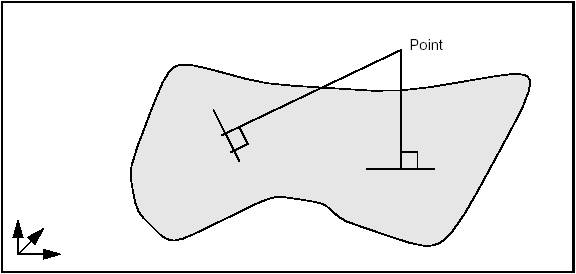Projection of normals from a point to a surface

Note that the surface does not have to be of Geom_RectangularTrimmedSurface type. The algorithm will function with any class inheriting Geom_Surface.

GeomAPI_ProjectPointOnSurf is instantiated as in the following example:

gp_Pnt P;
Handle (Geom_Surface) S = new Geom_BezierSurface(args);
GeomAPI_ProjectPointOnSurf Proj (P, S);

To restrict the search for normals within the given rectangular domain [U1, U2, V1, V2], use the constructor GeomAPI_ProjectPointOnSurf Proj (P, S, U1, U2, V1, V2)

The values of U1, U2, V1 and V2 lie at or within their maximum and minimum limits, i.e.:

Umin <= U1 < U2 <= Umax
Vmin <= V1 < V2 <= Vmax

Having thus created the GeomAPI_ProjectPointOnSurf object, you can interrogate it.

#### Calling the number of solution points

Standard_Integer NumSolutions = Proj.NbPoints();

#### Calling the location of a solution point

The solutions are indexed in a range from 1 to Proj.NbPoints(). The point corresponding to the given index may be found:

gp_Pnt Pn = Proj.Point(Index);

#### Calling the parameters of a solution point

For a given point corresponding to the given index:

Standard_Real U,V;
Proj.Parameters(Index, U, V);

#### Calling the distance between the start and end point

The distance between the initial point and a point corresponding to the given index may be found:

Standard_Real D = Projector.Distance(Index);

#### Calling the nearest solution point

This class offers a method, which returns the closest solution point to the starting point. This solution is accessed as follows:

gp_Pnt P1 = Proj.NearestPoint();

#### Calling the parameters of the nearest solution point

Standard_Real U,V;
Proj.LowerDistanceParameters (U, V);

#### Calling the minimum distance from a point to the surface

Standard_Real D = Proj.LowerDistance();

#### Redefined operators

Some operators have been redefined to help you find the nearest solution.

Standard_Real() returns the minimum distance from the point to the surface.

Standard_Real D = GeomAPI_ProjectPointOnSurf (P,S);

Standard_Integer() returns the number of solutions.

Standard_Integer N = GeomAPI_ProjectPointOnSurf (P,S);

gp_Pnt2d() returns the nearest solution point.

gp_Pnt P1 = GeomAPI_ProjectPointOnSurf (P,S);

Using these operators makes coding easier when you only need the nearest point. In this way,

GeomAPI_ProjectPointOnSurface Proj (P, S);
gp_Pnt P1 = Proj.NearestPoint();

can be written more concisely as:

gp_Pnt P1 = GeomAPI_ProjectPointOnSurface (P,S);

In the second case, however, no intermediate GeomAPI_ProjectPointOnSurf object is created, and it is impossible to access other solution points.

If you want to use the wider range of functionalities available from the Extrema package, a call to the Extrema() method will return the algorithmic object for calculating the extrema as follows:

Extrema_ExtPS& TheExtrema = Proj.Extrema();

### Switching from 2d and 3d Curves

The To2d and To3d methods are used to;

• build a 2d curve from a 3d Geom_Curve lying on a gp_Pln plane
• build a 3d curve from a Geom2d_Curve and a gp_Pln plane.

These methods are called as follows:

Handle(Geom2d_Curve) C2d = GeomAPI::To2d(C3d, Pln);
Handle(Geom_Curve) C3d = GeomAPI::To3d(C2d, Pln);

# Topological Tools

Open CASCADE Technology topological tools provide algorithms to

• Create wires from edges;
• Create faces from wires;
• Compute state of the shape relatively other shape;
• Orient shapes in container;
• Create new shapes from the existing ones;
• Build PCurves of edges on the faces;
• Check the validity of the shapes;
• Take the point in the face;
• Get the normal direction for the face.

## Creation of the faces from wireframe model

It is possible to create the planar faces from the arbitrary set of planar edges randomly located in 3D space. This feature might be useful if you need for instance to restore the shape from the wireframe model: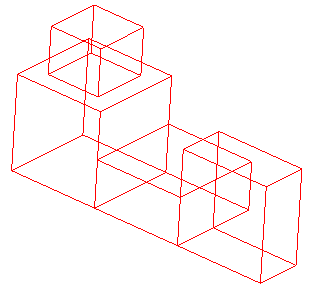Wireframe model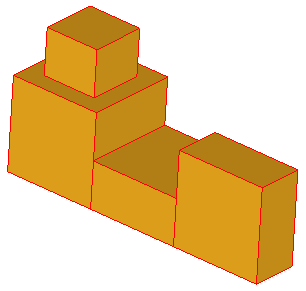Faces of the model

To make the faces from edges it is, firstly, necessary to create planar wires from the given edges and than create planar faces from each wire. The static methods BOPAlgo_Tools::EdgesToWires and BOPAlgo_Tools::WiresToFaces can be used for that:

TopoDS_Shape anEdges = ...; /* The input edges */
Standard_Real anAngTol = 1.e-8; /* The angular tolerance for distinguishing the planes in which the wires are located */
Standard_Boolean bShared = Standard_False; /* Defines whether the edges are shared or not */
//
TopoDS_Shape aWires; /* resulting wires */
Standard_Integer iErr = BOPAlgo_Tools::EdgesToWires(anEdges, aWires, bShared, anAngTol);
if (iErr) {
cout << "Error: Unable to build wires from given edges\n";
return;
}
//
TopoDS_Shape aFaces; /* resulting faces */
Standard_Boolean bDone = BOPAlgo_Tools::WiresToFaces(aWires, aFaces, anAngTol);
if (!bDone) {
cout << "Error: Unable to build faces from wires\n";
return;
}

These methods can also be used separately:

• BOPAlgo_Tools::EdgesToWires allows creating planar wires from edges. The input edges may be not shared, but the output wires will be sharing the coinciding vertices and edges. For this the intersection of the edges is performed. Although, it is possible to skip the intersection stage (if the input edges are already shared) by passing the corresponding flag into the method. The input edges are expected to be planar, but the method does not check it. Thus, if the input edges are not planar, the output wires will also be not planar. In general, the output wires are non-manifold and may contain free vertices, as well as multi-connected vertices.
• BOPAlgo_Tools::WiresToFaces allows creating planar faces from the planar wires. In general, the input wires are non-manifold and may be not closed, but should share the coinciding parts. The wires located in the same plane and completely included into other wires will create holes in the faces built from outer wires: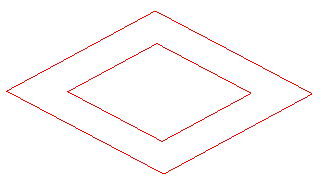Wireframe model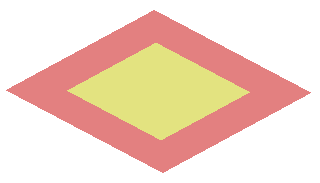Two faces (red face has a hole)

## Classification of the shapes

The following methods allow classifying the different shapes relatively other shapes:

• The variety of the BOPTools_AlgoTools::ComputState methods classify the vertex/edge/face relatively solid;
• BOPTools_AlgoTools::IsHole classifies wire relatively face;
• IntTools_Tools::ClassifyPointByFace classifies point relatively face.

## Orientation of the shapes in the container

The following methods allow reorienting shapes in the containers:

• BOPTools_AlgoTools::OrientEdgesOnWire correctly orients edges on the wire;
• BOPTools_AlgoTools::OrientFacesOnShell correctly orients faces on the shell.

## Making new shapes

The following methods allow creating new shapes from the existing ones:

• The variety of the BOPTools_AlgoTools::MakeNewVertex creates the new vertices from other vertices and edges;
• BOPTools_AlgoTools::MakeSplitEdge splits the edge by the given parameters.

## Building PCurves

The following methods allow building PCurves of edges on faces:

• BOPTools_AlgoTools::BuildPCurveForEdgeOnFace computes PCurve for the edge on the face;
• BOPTools_AlgoTools::BuildPCurveForEdgeOnPlane and BOPTools_AlgoTools::BuildPCurveForEdgesOnPlane allow building PCurves for edges on the planar face;
• BOPTools_AlgoTools::AttachExistingPCurve takes PCurve on the face from one edge and attach this PCurve to other edge coinciding with the first one.

## Checking the validity of the shapes

The following methods allow checking the validity of the shapes:

• BOPTools_AlgoTools::IsMicroEdge detects the small edges;
• BOPTools_AlgoTools::ComputeTolerance computes the correct tolerance of the edge on the face;
• BOPTools_AlgoTools::CorrectShapeTolerances and BOPTools_AlgoTools::CorrectTolerances allow correcting the tolerances of the sub-shapes.
• BRepLib::FindValidRange finds a range of 3d curve of the edge not covered by tolerance spheres of vertices.

## Taking a point inside the face

The following methods allow taking a point located inside the face:

• The variety of the BOPTools_AlgoTools3D::PointNearEdge allows getting a point inside the face located near the edge;
• BOPTools_AlgoTools3D::PointInFace allows getting a point inside the face.

## Getting normal for the face

The following methods allow getting the normal direction for the face/surface:

• BOPTools_AlgoTools3D::GetNormalToSurface computes the normal direction for the surface in the given point defined by UV parameters;
• BOPTools_AlgoTools3D::GetNormalToFaceOnEdge computes the normal direction for the face in the point located on the edge of the face;
• BOPTools_AlgoTools3D::GetApproxNormalToFaceOnEdge computes the normal direction for the face in the point located near the edge of the face.

# The Topology API

The Topology API of Open CASCADE Technology (OCCT) includes the following six packages:

• BRepAlgoAPI
• BRepBuilderAPI
• BRepFilletAPI
• BRepFeat
• BRepOffsetAPI
• BRepPrimAPI

The classes provided by the API have the following features:

• The constructors of classes provide different construction methods;
• The class retains different tools used to build objects as fields;
• The class provides a casting method to obtain the result automatically with a function-like call.

Let us use the class BRepBuilderAPI_MakeEdge to create a linear edge from two points.

gp_Pnt P1(10,0,0), P2(20,0,0);
TopoDS_Edge E = BRepBuilderAPI_MakeEdge(P1,P2);

This is the simplest way to create edge E from two points P1, P2, but the developer can test for errors when he is not as confident of the data as in the previous example.

#include <gp_Pnt.hxx>
#include <TopoDS_Edge.hxx>
#include <BRepBuilderAPI_MakeEdge.hxx>
void EdgeTest()
{
gp_Pnt P1;
gp_Pnt P2;
BRepBuilderAPI_MakeEdge ME(P1,P2);
if (!ME.IsDone())
{
// doing ME.Edge() or E = ME here
// would raise StdFail_NotDone
Standard_DomainError::Raise
(“ProcessPoints::Failed to createan edge”);
}
TopoDS_Edge E = ME;
}

In this example an intermediary object ME has been introduced. This can be tested for the completion of the function before accessing the result. More information on error handling in the topology programming interface can be found in the next section.

BRepBuilderAPI_MakeEdge provides valuable information. For example, when creating an edge from two points, two vertices have to be created from the points. Sometimes you may be interested in getting these vertices quickly without exploring the new edge. Such information can be provided when using a class. The following example shows a function creating an edge and two vertices from two points.

void MakeEdgeAndVertices(const gp_Pnt& P1,
const gp_Pnt& P2,
TopoDS_Edge& E,
TopoDS_Vertex& V1,
TopoDS_Vertex& V2)
{
BRepBuilderAPI_MakeEdge ME(P1,P2);
if (!ME.IsDone()) {
Standard_DomainError::Raise
(“MakeEdgeAndVerices::Failed to create an edge”);
}
E = ME;
V1 = ME.Vextex1();
V2 = ME.Vertex2();

The class BRepBuilderAPI_MakeEdge provides two methods Vertex1 and Vertex2, which return two vertices used to create the edge.

How can BRepBuilderAPI_MakeEdge be both a function and a class? It can do this because it uses the casting capabilities of C++. The BRepBuilderAPI_MakeEdge class has a method called Edge; in the previous example the line E = ME could have been written.

E = ME.Edge();

This instruction tells the C++ compiler that there is an implicit casting of a BRepBuilderAPI_MakeEdge into a TopoDS_Edge using the Edge method. It means this method is automatically called when a BRepBuilderAPI_MakeEdge is found where a TopoDS_Edge is required.

This feature allows you to provide classes, which have the simplicity of function calls when required and the power of classes when advanced processing is necessary. All the benefits of this approach are explained when describing the topology programming interface classes.

## Error Handling in the Topology API

A method can report an error in the two following situations:

• The data or arguments of the method are incorrect, i.e. they do not respect the restrictions specified by the methods in its specifications. Typical example: creating a linear edge from two identical points is likely to lead to a zero divide when computing the direction of the line.
• Something unexpected happened. This situation covers every error not included in the first category. Including: interruption, programming errors in the method or in another method called by the first method, bad specifications of the arguments (i.e. a set of arguments that was not expected to fail).

The second situation is supposed to become increasingly exceptional as a system is debugged and it is handled by the exception mechanism. Using exceptions avoids handling error statuses in the call to a method: a very cumbersome style of programming.

In the first situation, an exception is also supposed to be raised because the calling method should have verified the arguments and if it did not do so, there is a bug. For example, if before calling MakeEdge you are not sure that the two points are non-identical, this situation must be tested.

Making those validity checks on the arguments can be tedious to program and frustrating as you have probably correctly surmised that the method will perform the test twice. It does not trust you. As the test involves a great deal of computation, performing it twice is also time-consuming.

Consequently, you might be tempted to adopt the highly inadvisable style of programming illustrated in the following example:

#include <Standard_ErrorHandler.hxx>
try {
TopoDS_Edge E = BRepBuilderAPI_MakeEdge(P1,P2);
// go on with the edge
}
catch {
// process the error.
}

To help the user, the Topology API classes only raise the exception StdFail_NotDone. Any other exception means that something happened which was unforeseen in the design of this API.

The NotDone exception is only raised when the user tries to access the result of the computation and the original data is corrupted. At the construction of the class instance, if the algorithm cannot be completed, the internal flag NotDone is set. This flag can be tested and in some situations a more complete description of the error can be queried. If the user ignores the NotDone status and tries to access the result, an exception is raised.

BRepBuilderAPI_MakeEdge ME(P1,P2);
if (!ME.IsDone()) {
// doing ME.Edge() or E = ME here
// would raise StdFail_NotDone
Standard_DomainError::Raise
(“ProcessPoints::Failed to create an edge”);
}
TopoDS_Edge E = ME;

# Standard Topological Objects

The following standard topological objects can be created:

• Vertices
• Edges
• Faces
• Wires
• Polygonal wires
• Shells
• Solids.

There are two root classes for their construction and modification:

• The deferred class BRepBuilderAPI_MakeShape is the root of all BRepBuilderAPI classes, which build shapes. It inherits from the class BRepBuilderAPI_Command and provides a field to store the constructed shape.
• The deferred class BRepBuilderAPI_ModifyShape is used as a root for the shape modifications. It inherits BRepBuilderAPI_MakeShape and implements the methods used to trace the history of all sub-shapes.

## Vertex

BRepBuilderAPI_MakeVertex creates a new vertex from a 3D point from gp.

gp_Pnt P(0,0,10);
TopoDS_Vertex V = BRepBuilderAPI_MakeVertex(P);

This class always creates a new vertex and has no other methods.

## Edge

### Basic edge construction method

Use BRepBuilderAPI_MakeEdge to create from a curve and vertices. The basic method constructs an edge from a curve, two vertices, and two parameters.

Handle(Geom_Curve) C = ...; // a curve
TopoDS_Vertex V1 = ...,V2 = ...;// two Vertices
Standard_Real p1 = ..., p2 = ..;// two parameters
TopoDS_Edge E = BRepBuilderAPI_MakeEdge(C,V1,V2,p1,p2);

where C is the domain of the edge; V1 is the first vertex oriented FORWARD; V2 is the second vertex oriented REVERSED; p1 and p2 are the parameters for the vertices V1 and V2 on the curve. The default tolerance is associated with this edge.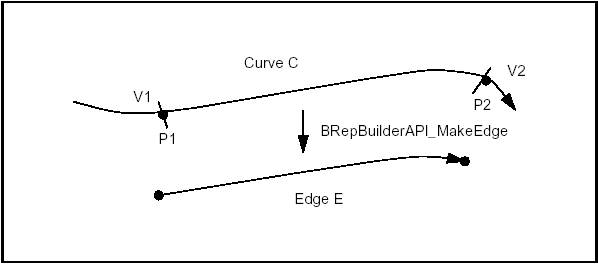Basic Edge Construction

The following rules apply to the arguments:

The curve

• Must not be a Null Handle.
• If the curve is a trimmed curve, the basis curve is used.

The vertices

• Can be null shapes. When V1 or V2 is Null the edge is open in the corresponding direction and the corresponding parameter p1 or p2 must be infinite (i.e p1 is RealFirst(), p2 is RealLast()).
• Must be different vertices if they have different 3d locations and identical vertices if they have the same 3d location (identical vertices are used when the curve is closed).

The parameters

• Must be increasing and in the range of the curve, i.e.:
C->FirstParameter() <= p1 < p2 <= C->LastParameter()
• If the parameters are decreasing, the Vertices are switched, i.e. V2 becomes V1 and V1 becomes V2.
• On a periodic curve the parameters p1 and p2 are adjusted by adding or subtracting the period to obtain p1 in the range of the curve and p2 in the range p1 < p2 <= p1+ Period. So on a parametric curve p2 can be greater than the second parameter, see the figure below.
• Can be infinite but the corresponding vertex must be Null (see above).
• The distance between the Vertex 3d location and the point evaluated on the curve with the parameter must be lower than the default precision.

The figure below illustrates two special cases, a semi-infinite edge and an edge on a periodic curve.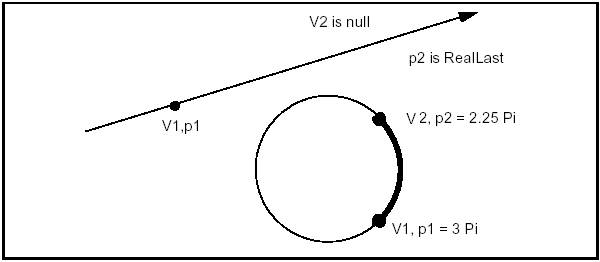Infinite and Periodic Edges

### Supplementary edge construction methods

There exist supplementary edge construction methods derived from the basic one.

BRepBuilderAPI_MakeEdge class provides methods, which are all simplified calls of the previous one:

• The parameters can be omitted. They are computed by projecting the vertices on the curve.
• 3d points (Pnt from gp) can be given in place of vertices. Vertices are created from the points. Giving vertices is useful when creating connected vertices.
• The vertices or points can be omitted if the parameters are given. The points are computed by evaluating the parameters on the curve.
• The vertices or points and the parameters can be omitted. The first and the last parameters of the curve are used.

The five following methods are thus derived from the basic construction:

Handle(Geom_Curve) C = ...; // a curve
TopoDS_Vertex V1 = ...,V2 = ...;// two Vertices
Standard_Real p1 = ..., p2 = ..;// two parameters
gp_Pnt P1 = ..., P2 = ...;// two points
TopoDS_Edge E;
// project the vertices on the curve
E = BRepBuilderAPI_MakeEdge(C,V1,V2);
// Make vertices from points
E = BRepBuilderAPI_MakeEdge(C,P1,P2,p1,p2);
// Make vertices from points and project them
E = BRepBuilderAPI_MakeEdge(C,P1,P2);
// Computes the points from the parameters
E = BRepBuilderAPI_MakeEdge(C,p1,p2);
// Make an edge from the whole curve
E = BRepBuilderAPI_MakeEdge(C);

Six methods (the five above and the basic method) are also provided for curves from the gp package in place of Curve from Geom. The methods create the corresponding Curve from Geom and are implemented for the following classes:

gp_Lin creates a Geom_Line gp_Circ creates a Geom_Circle gp_Elips creates a Geom_Ellipse gp_Hypr creates a Geom_Hyperbola gp_Parab creates a Geom_Parabola

There are also two methods to construct edges from two vertices or two points. These methods assume that the curve is a line; the vertices or points must have different locations.

TopoDS_Vertex V1 = ...,V2 = ...;// two Vertices
gp_Pnt P1 = ..., P2 = ...;// two points
TopoDS_Edge E;
// linear edge from two vertices
E = BRepBuilderAPI_MakeEdge(V1,V2);
// linear edge from two points
E = BRepBuilderAPI_MakeEdge(P1,P2);

### Other information and error status

The class BRepBuilderAPI_MakeEdge can provide extra information and return an error status.

If BRepBuilderAPI_MakeEdge is used as a class, it can provide two vertices. This is useful when the vertices were not provided as arguments, for example when the edge was constructed from a curve and parameters. The two methods Vertex1 and Vertex2 return the vertices. Note that the returned vertices can be null if the edge is open in the corresponding direction.

The Error method returns a term of the BRepBuilderAPI_EdgeError enumeration. It can be used to analyze the error when IsDone method returns False. The terms are:

• EdgeDone – No error occurred, IsDone returns True.
• PointProjectionFailed – No parameters were given, but the projection of the 3D points on the curve failed. This happens if the point distance to the curve is greater than the precision.
• ParameterOutOfRange – The given parameters are not in the range C->FirstParameter(), C->LastParameter()
• DifferentPointsOnClosedCurve – The two vertices or points have different locations but they are the extremities of a closed curve.
• PointWithInfiniteParameter – A finite coordinate point was associated with an infinite parameter (see the Precision package for a definition of infinite values).
• DifferentsPointAndParameter – The distance of the 3D point and the point evaluated on the curve with the parameter is greater than the precision.
• LineThroughIdenticPoints – Two identical points were given to define a line (construction of an edge without curve), gp::Resolution is used to test confusion .

The following example creates a rectangle centered on the origin of dimensions H, L with fillets of radius R. The edges and the vertices are stored in the arrays theEdges and theVertices. We use class Array1OfShape (i.e. not arrays of edges or vertices). See the image below.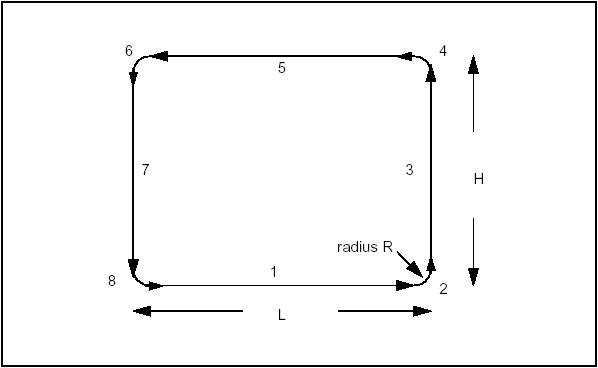Creating a Wire
#include <BRepBuilderAPI_MakeEdge.hxx>
#include <TopoDS_Shape.hxx>
#include <gp_Circ.hxx>
#include <gp.hxx>
#include <TopoDS_Wire.hxx>
#include <TopTools_Array1OfShape.hxx>
#include <BRepBuilderAPI_MakeWire.hxx>
// Use MakeArc method to make an edge and two vertices
void MakeArc(Standard_Real x,Standard_Real y,
Standard_Real R,
Standard_Real ang,
TopoDS_Shape& E,
TopoDS_Shape& V1,
TopoDS_Shape& V2)
{
gp_Ax2 Origin = gp::XOY();
gp_Vec Offset(x, y, 0.);
Origin.Translate(Offset);
BRepBuilderAPI_MakeEdge
ME(gp_Circ(Origin,R), ang, ang+PI/2);
E = ME;
V1 = ME.Vertex1();
V2 = ME.Vertex2();
}
TopoDS_Wire MakeFilletedRectangle(const Standard_Real H,
const Standard_Real L,
const Standard_Real R)
{
TopTools_Array1OfShape theEdges(1,8);
TopTools_Array1OfShape theVertices(1,8);
// First create the circular edges and the vertices
// using the MakeArc function described above.
void MakeArc(Standard_Real, Standard_Real,
Standard_Real, Standard_Real,
TopoDS_Shape&, TopoDS_Shape&, TopoDS_Shape&);
Standard_Real x = L/2 - R, y = H/2 - R;
MakeArc(x,-y,R,3.*PI/2.,theEdges(2),theVertices(2),
theVertices(3));
MakeArc(x,y,R,0.,theEdges(4),theVertices(4),
theVertices(5));
MakeArc(-x,y,R,PI/2.,theEdges(6),theVertices(6),
theVertices(7));
MakeArc(-x,-y,R,PI,theEdges(8),theVertices(8),
theVertices(1));
// Create the linear edges
for (Standard_Integer i = 1; i <= 7; i += 2)
{
theEdges(i) = BRepBuilderAPI_MakeEdge
(TopoDS::Vertex(theVertices(i)),TopoDS::Vertex
(theVertices(i+1)));
}
// Create the wire using the BRepBuilderAPI_MakeWire
BRepBuilderAPI_MakeWire MW;
for (i = 1; i <= 8; i++)
{
}
return MW.Wire();
}

## Edge 2D

Use BRepBuilderAPI_MakeEdge2d class to make edges on a working plane from 2d curves. The working plane is a default value of the BRepBuilderAPI package (see the Plane methods).

BRepBuilderAPI_MakeEdge2d class is strictly similar to BRepBuilderAPI_MakeEdge, but it uses 2D geometry from gp and Geom2d instead of 3D geometry.

## Polygon

BRepBuilderAPI_MakePolygon class is used to build polygonal wires from vertices or points. Points are automatically changed to vertices as in BRepBuilderAPI_MakeEdge.

The basic usage of BRepBuilderAPI_MakePolygon is to create a wire by adding vertices or points using the Add method. At any moment, the current wire can be extracted. The close method can be used to close the current wire. In the following example, a closed wire is created from an array of points.

#include <TopoDS_Wire.hxx>
#include <BRepBuilderAPI_MakePolygon.hxx>
#include <TColgp_Array1OfPnt.hxx>
TopoDS_Wire ClosedPolygon(const TColgp_Array1OfPnt& Points)
{
BRepBuilderAPI_MakePolygon MP;
for(Standard_Integer i=Points.Lower();i=Points.Upper();i++)
{
}
MP.Close();
return MP;
}

Short-cuts are provided for 2, 3, or 4 points or vertices. Those methods have a Boolean last argument to tell if the polygon is closed. The default value is False.

Two examples:

Example of a closed triangle from three vertices:

TopoDS_Wire W = BRepBuilderAPI_MakePolygon(V1,V2,V3,Standard_True);

Example of an open polygon from four points:

TopoDS_Wire W = BRepBuilderAPI_MakePolygon(P1,P2,P3,P4);

BRepBuilderAPI_MakePolygon class maintains a current wire. The current wire can be extracted at any moment and the construction can proceed to a longer wire. After each point insertion, the class maintains the last created edge and vertex, which are returned by the methods Edge, FirstVertex and LastVertex.

When the added point or vertex has the same location as the previous one it is not added to the current wire but the most recently created edge becomes Null. The Added method can be used to test this condition. The MakePolygon class never raises an error. If no vertex has been added, the Wire is Null. If two vertices are at the same location, no edge is created.

## Face

Use BRepBuilderAPI_MakeFace class to create a face from a surface and wires. An underlying surface is constructed from a surface and optional parametric values. Wires can be added to the surface. A planar surface can be constructed from a wire. An error status can be returned after face construction.

### Basic face construction method

A face can be constructed from a surface and four parameters to determine a limitation of the UV space. The parameters are optional, if they are omitted the natural bounds of the surface are used. Up to four edges and vertices are created with a wire. No edge is created when the parameter is infinite.

Handle(Geom_Surface) S = ...; // a surface
Standard_Real umin,umax,vmin,vmax; // parameters
TopoDS_Face F = BRepBuilderAPI_MakeFace(S,umin,umax,vmin,vmax);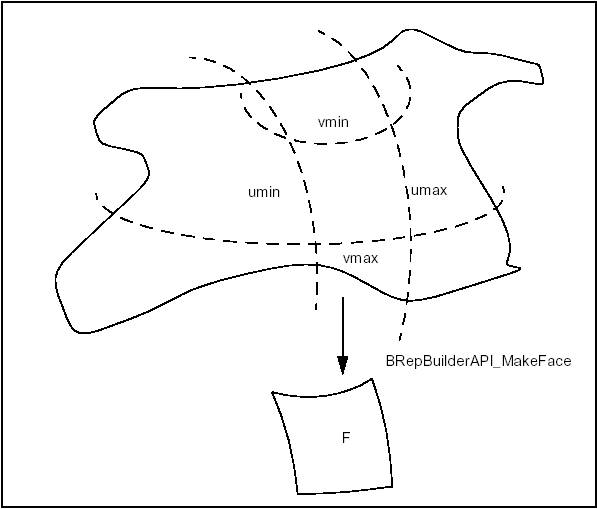Basic Face Construction

To make a face from the natural boundary of a surface, the parameters are not required:

Handle(Geom_Surface) S = ...; // a surface
TopoDS_Face F = BRepBuilderAPI_MakeFace(S);

Constraints on the parameters are similar to the constraints in BRepBuilderAPI_MakeEdge.

• umin,umax (vmin,vmax) must be in the range of the surface and must be increasing.
• On a U (V) periodic surface umin and umax (vmin,vmax) are adjusted.
• umin, umax, vmin, vmax can be infinite. There will be no edge in the corresponding direction.

### Supplementary face construction methods

The two basic constructions (from a surface and from a surface and parameters) are implemented for all gp package surfaces, which are transformed in the corresponding Surface from Geom.

gp package surface Geom package surface
gp_Pln Geom_Plane
gp_Cylinder Geom_CylindricalSurface
gp_Cone creates a Geom_ConicalSurface
gp_Sphere Geom_SphericalSurface
gp_Torus Geom_ToroidalSurface

Once a face has been created, a wire can be added using the Add method. For example, the following code creates a cylindrical surface and adds a wire.

gp_Cylinder C = ..; // a cylinder
TopoDS_Wire W = ...;// a wire
BRepBuilderAPI_MakeFace MF(C);
TopoDS_Face F = MF;

More than one wire can be added to a face, provided that they do not cross each other and they define only one area on the surface. (Note that this is not checked). The edges on a Face must have a parametric curve description.

If there is no parametric curve for an edge of the wire on the Face it is computed by projection.

For one wire, a simple syntax is provided to construct the face from the surface and the wire. The above lines could be written:

TopoDS_Face F = BRepBuilderAPI_MakeFace(C,W);

A planar face can be created from only a wire, provided this wire defines a plane. For example, to create a planar face from a set of points you can use BRepBuilderAPI_MakePolygon and BRepBuilderAPI_MakeFace.

#include <TopoDS_Face.hxx>
#include <TColgp_Array1OfPnt.hxx>
#include <BRepBuilderAPI_MakePolygon.hxx>
#include <BRepBuilderAPI_MakeFace.hxx>
TopoDS_Face PolygonalFace(const TColgp_Array1OfPnt& thePnts)
{
BRepBuilderAPI_MakePolygon MP;
for(Standard_Integer i=thePnts.Lower();
i<=thePnts.Upper(); i++)
{
}
MP.Close();
TopoDS_Face F = BRepBuilderAPI_MakeFace(MP.Wire());
return F;
}

The last use of MakeFace is to copy an existing face to add new wires. For example, the following code adds a new wire to a face:

TopoDS_Face F = ...; // a face
TopoDS_Wire W = ...; // a wire
F = BRepBuilderAPI_MakeFace(F,W);

To add more than one wire an instance of the BRepBuilderAPI_MakeFace class can be created with the face and the first wire and the new wires inserted with the Add method.

### Error status

The Error method returns an error status, which is a term from the BRepBuilderAPI_FaceError enumeration.

• FaceDone – no error occurred.
• NoFace – no initialization of the algorithm; an empty constructor was used.
• NotPlanar – no surface was given and the wire was not planar.
• CurveProjectionFailed – no curve was found in the parametric space of the surface for an edge.
• ParametersOutOfRange – the parameters umin, umax, vmin, vmax are out of the surface.

## Wire

The wire is a composite shape built not from a geometry, but by the assembly of edges. BRepBuilderAPI_MakeWire class can build a wire from one or more edges or connect new edges to an existing wire.

Up to four edges can be used directly, for example:

TopoDS_Wire W = BRepBuilderAPI_MakeWire(E1,E2,E3,E4);

For a higher or unknown number of edges the Add method must be used; for example, to build a wire from an array of shapes (to be edges).

TopTools_Array1OfShapes theEdges;
BRepBuilderAPI_MakeWire MW;
for (Standard_Integer i = theEdge.Lower();
i <= theEdges.Upper(); i++)
TopoDS_Wire W = MW;

The class can be constructed with a wire. A wire can also be added. In this case, all the edges of the wires are added. For example to merge two wires:

#include <TopoDS_Wire.hxx>
#include <BRepBuilderAPI_MakeWire.hxx>
TopoDS_Wire MergeWires (const TopoDS_Wire& W1,
const TopoDS_Wire& W2)
{
BRepBuilderAPI_MakeWire MW(W1);
return MW;
}

BRepBuilderAPI_MakeWire class connects the edges to the wire. When a new edge is added if one of its vertices is shared with the wire it is considered as connected to the wire. If there is no shared vertex, the algorithm searches for a vertex of the edge and a vertex of the wire, which are at the same location (the tolerances of the vertices are used to test if they have the same location). If such a pair of vertices is found, the edge is copied with the vertex of the wire in place of the original vertex. All the vertices of the edge can be exchanged for vertices from the wire. If no connection is found the wire is considered to be disconnected. This is an error.

BRepBuilderAPI_MakeWire class can return the last edge added to the wire (Edge method). This edge can be different from the original edge if it was copied.

The Error method returns a term of the BRepBuilderAPI_WireError enumeration: WireDone – no error occurred. EmptyWire – no initialization of the algorithm, an empty constructor was used. DisconnectedWire – the last added edge was not connected to the wire. NonManifoldWire – the wire with some singularity.

## Shell

The shell is a composite shape built not from a geometry, but by the assembly of faces. Use BRepBuilderAPI_MakeShell class to build a Shell from a set of Faces. What may be important is that each face should have the required continuity. That is why an initial surface is broken up into faces.

## Solid

The solid is a composite shape built not from a geometry, but by the assembly of shells. Use BRepBuilderAPI_MakeSolid class to build a Solid from a set of Shells. Its use is similar to the use of the MakeWire class: shells are added to the solid in the same way that edges are added to the wire in MakeWire.

# Object Modification

## Transformation

BRepBuilderAPI_Transform class can be used to apply a transformation to a shape (see class gp_Trsf). The methods have a boolean argument to copy or share the original shape, as long as the transformation allows (it is only possible for direct isometric transformations). By default, the original shape is shared.

The following example deals with the rotation of shapes.

TopoDS_Shape myShape1 = ...;
// The original shape 1
TopoDS_Shape myShape2 = ...;
// The original shape2
gp_Trsf T;
T.SetRotation(gp_Ax1(gp_Pnt(0.,0.,0.),gp_Vec(0.,0.,1.)),
2.*PI/5.);
BRepBuilderAPI_Transformation theTrsf(T);
theTrsf.Perform(myShape1);
TopoDS_Shape myNewShape1 = theTrsf.Shape()
theTrsf.Perform(myShape2,Standard_True);
// Here duplication is forced
TopoDS_Shape myNewShape2 = theTrsf.Shape()

## Duplication

Use the BRepBuilderAPI_Copy class to duplicate a shape. A new shape is thus created. In the following example, a solid is copied:

TopoDS Solid MySolid;
....// Creates a solid
TopoDS_Solid myCopy = BRepBuilderAPI_Copy(mySolid);

# Primitives

The BRepPrimAPI package provides an API (Application Programming Interface) for construction of primitives such as:

• Boxes;
• Cones;
• Cylinders;
• Prisms.

It is possible to create partial solids, such as a sphere limited by longitude. In real models, primitives can be used for easy creation of specific sub-parts.

• Construction by sweeping along a profile:
• Linear;
• Rotational (through an angle of rotation).

Sweeps are objects obtained by sweeping a profile along a path. The profile can be any topology and the path is usually a curve or a wire. The profile generates objects according to the following rules:

• Vertices generate Edges
• Edges generate Faces.
• Wires generate Shells.
• Faces generate Solids.
• Shells generate Composite Solids.

It is not allowed to sweep Solids and Composite Solids. Swept constructions along complex profiles such as BSpline curves also available in the BRepOffsetAPI package. This API provides simple, high level calls for the most common operations.

## Making Primitives

### Box

The class BRepPrimAPI_MakeBox allows building a parallelepiped box. The result is either a Shell or a Solid. There are four ways to build a box:

• From three dimensions dx, dy and dz. The box is parallel to the axes and extends for [0,dx] [0,dy] [0,dz] .
• From a point and three dimensions. The same as above but the point is the new origin.
• From two points, the box is parallel to the axes and extends on the intervals defined by the coordinates of the two points.
• From a system of axes gp_Ax2 and three dimensions. Same as the first way but the box is parallel to the given system of axes.

An error is raised if the box is flat in any dimension using the default precision. The following code shows how to create a box:

TopoDS_Solid theBox = BRepPrimAPI_MakeBox(10.,20.,30.);

The four methods to build a box are shown in the figure: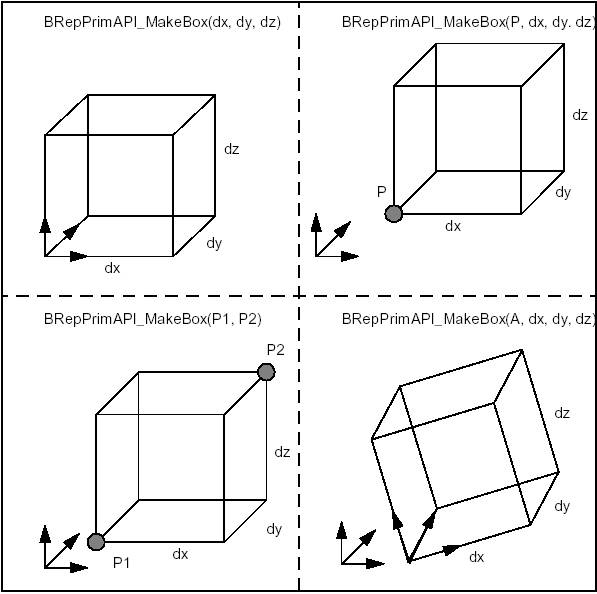Making Boxes

### Wedge

BRepPrimAPI_MakeWedge class allows building a wedge, which is a slanted box, i.e. a box with angles. The wedge is constructed in much the same way as a box i.e. from three dimensions dx,dy,dz plus arguments or from an axis system, three dimensions, and arguments.

The following figure shows two ways to build wedges. One is to add a dimension ltx, which is the length in x of the face at dy. The second is to add xmin, xmax, zmin and zmax to describe the face at dy.

The first method is a particular case of the second with xmin = 0, xmax = ltx, zmin = 0, zmax = dz. To make a centered pyramid you can use xmin = xmax = dx / 2, zmin = zmax = dz / 2.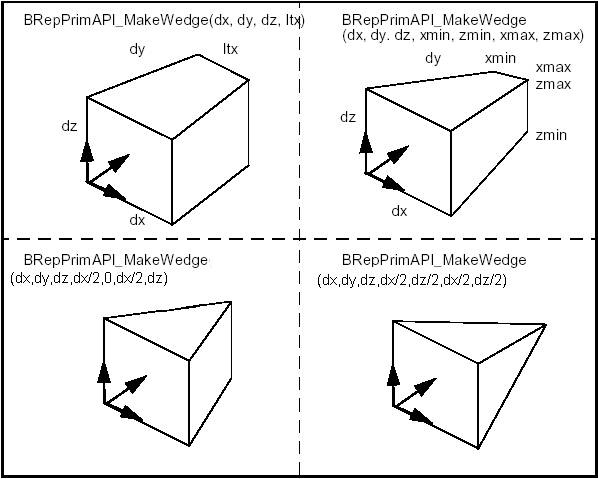Making Wedges

### Rotation object

BRepPrimAPI_MakeOneAxis is a deferred class used as a root class for all classes constructing rotational primitives. Rotational primitives are created by rotating a curve around an axis. They cover the cylinder, the cone, the sphere, the torus, and the revolution, which provides all other curves.

The particular constructions of these primitives are described, but they all have some common arguments, which are:

• A system of coordinates, where the Z axis is the rotation axis..
• An angle in the range [0,2*PI].
• A vmin, vmax parameter range on the curve.

The result of the OneAxis construction is a Solid, a Shell, or a Face. The face is the face covering the rotational surface. Remember that you will not use the OneAxis directly but one of the derived classes, which provide improved constructions. The following figure illustrates the OneAxis arguments.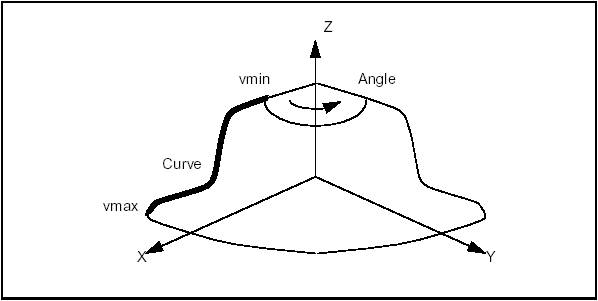MakeOneAxis arguments

### Cylinder

BRepPrimAPI_MakeCylinder class allows creating cylindrical primitives. A cylinder is created either in the default coordinate system or in a given coordinate system gp_Ax2. There are two constructions:

• Radius and height, to build a full cylinder.
• Radius, height and angle to build a portion of a cylinder.

The following code builds the cylindrical face of the figure, which is a quarter of cylinder along the Y axis with the origin at X,Y,Z the length of DY and radius R.

Standard_Real X = 20, Y = 10, Z = 15, R = 10, DY = 30;
// Make the system of coordinates
gp_Ax2 axes = gp::ZOX();
axes.Translate(gp_Vec(X,Y,Z));
TopoDS_Face F =
BRepPrimAPI_MakeCylinder(axes,R,DY,PI/2.);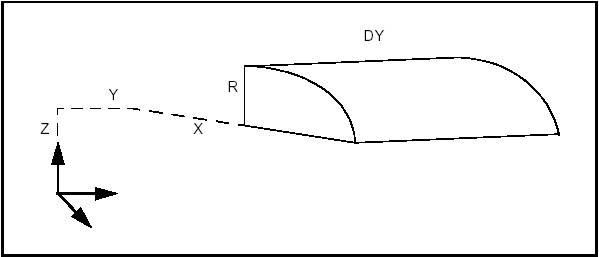Cylinder

### Cone

BRepPrimAPI_MakeCone class allows creating conical primitives. Like a cylinder, a cone is created either in the default coordinate system or in a given coordinate system (gp_Ax2). There are two constructions:

• Two radii and height, to build a full cone. One of the radii can be null to make a sharp cone.
• Radii, height and angle to build a truncated cone.

The following code builds the solid cone of the figure, which is located in the default system with radii R1 and R2 and height H.

Standard_Real R1 = 30, R2 = 10, H = 15;
TopoDS_Solid S = BRepPrimAPI_MakeCone(R1,R2,H);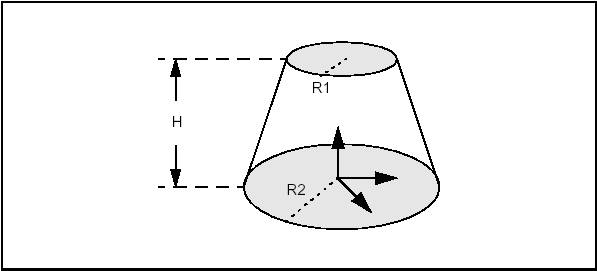Cone

### Sphere

BRepPrimAPI_MakeSphere class allows creating spherical primitives. Like a cylinder, a sphere is created either in the default coordinate system or in a given coordinate system gp_Ax2. There are four constructions:

• From a radius – builds a full sphere.
• From a radius and an angle – builds a lune (digon).
• From a radius and two angles – builds a wraparound spherical segment between two latitudes. The angles a1 and a2 must follow the relation: PI/2 <= a1 < a2 <= PI/2 .
• From a radius and three angles – a combination of two previous methods builds a portion of spherical segment.

The following code builds four spheres from a radius and three angles.

Standard_Real R = 30, ang =
PI/2, a1 = -PI/2.3, a2 = PI/4;
TopoDS_Solid S1 = BRepPrimAPI_MakeSphere(R);
TopoDS_Solid S2 = BRepPrimAPI_MakeSphere(R,ang);
TopoDS_Solid S3 = BRepPrimAPI_MakeSphere(R,a1,a2);
TopoDS_Solid S4 = BRepPrimAPI_MakeSphere(R,a1,a2,ang);

Note that we could equally well choose to create Shells instead of Solids.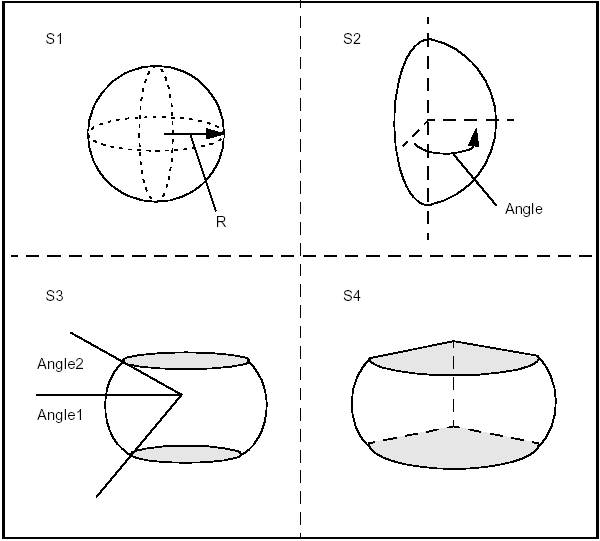Examples of Spheres

### Torus

BRepPrimAPI_MakeTorus class allows creating toroidal primitives. Like the other primitives, a torus is created either in the default coordinate system or in a given coordinate system gp_Ax2. There are four constructions similar to the sphere constructions:

• Two radii – builds a full torus.
• Two radii and an angle – builds an angular torus segment.
• Two radii and two angles – builds a wraparound torus segment between two radial planes. The angles a1, a2 must follow the relation 0 < a2 - a1 < 2*PI.
• Two radii and three angles – a combination of two previous methods builds a portion of torus segment.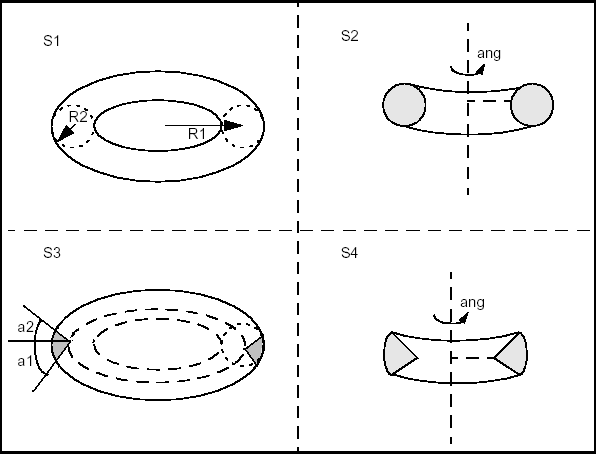Examples of Tori

The following code builds four toroidal shells from two radii and three angles.

Standard_Real R1 = 30, R2 = 10, ang = PI, a1 = 0,
a2 = PI/2;
TopoDS_Shell S1 = BRepPrimAPI_MakeTorus(R1,R2);
TopoDS_Shell S2 = BRepPrimAPI_MakeTorus(R1,R2,ang);
TopoDS_Shell S3 = BRepPrimAPI_MakeTorus(R1,R2,a1,a2);
TopoDS_Shell S4 =
BRepPrimAPI_MakeTorus(R1,R2,a1,a2,ang);

Note that we could equally well choose to create Solids instead of Shells.

### Revolution

BRepPrimAPI_MakeRevolution class allows building a uniaxial primitive from a curve. As other uniaxial primitives it can be created in the default coordinate system or in a given coordinate system.

The curve can be any Geom_Curve, provided it is planar and lies in the same plane as the Z-axis of local coordinate system. There are four modes of construction:

• From a curve, use the full curve and make a full rotation.
• From a curve and an angle of rotation.
• From a curve and two parameters to trim the curve. The two parameters must be growing and within the curve range.
• From a curve, two parameters, and an angle. The two parameters must be growing and within the curve range.

## Sweeping: Prism, Revolution and Pipe

### Sweeping

Sweeps are the objects you obtain by sweeping a profile along a path. The profile can be of any topology. The path is usually a curve or a wire. The profile generates objects according to the following rules:

• Vertices generate Edges
• Edges generate Faces.
• Wires generate Shells.
• Faces generate Solids.
• Shells generate Composite Solids

It is forbidden to sweep Solids and Composite Solids. A Compound generates a Compound with the sweep of all its elements.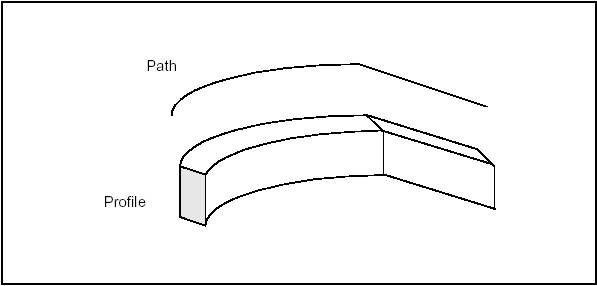Generating a sweep

BRepPrimAPI_MakeSweep class is a deferred class used as a root of the the following sweep classes:

• BRepPrimAPI_MakePrism – produces a linear sweep
• BRepPrimAPI_MakeRevol – produces a rotational sweep
• BRepPrimAPI_MakePipe – produces a general sweep.

### Prism

BRepPrimAPI_MakePrism class allows creating a linear prism from a shape and a vector or a direction.

• A vector allows creating a finite prism;
• A direction allows creating an infinite or semi-infinite prism. The semi-infinite or infinite prism is toggled by a Boolean argument. All constructors have a boolean argument to copy the original shape or share it (by default).

The following code creates a finite, an infinite and a semi-infinite solid using a face, a direction and a length.

TopoDS_Face F = ..; // The swept face
gp_Dir direc(0,0,1);
Standard_Real l = 10;
// create a vector from the direction and the length
gp_Vec v = direc;
v *= l;
TopoDS_Solid P1 = BRepPrimAPI_MakePrism(F,v);
// finite
TopoDS_Solid P2 = BRepPrimAPI_MakePrism(F,direc);
// infinite
TopoDS_Solid P3 = BRepPrimAPI_MakePrism(F,direc,Standard_False);
// semi-infinite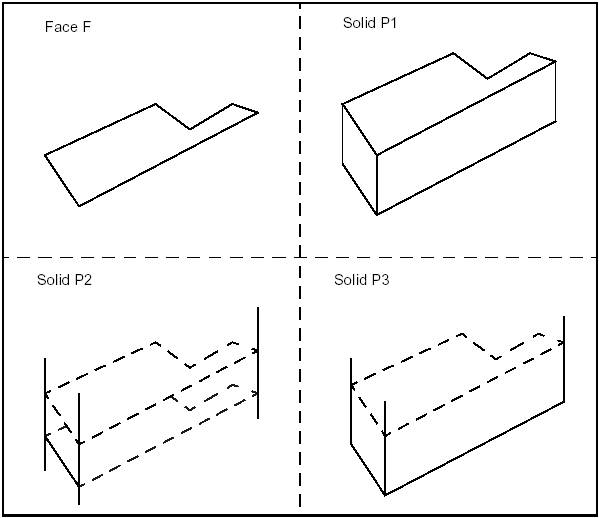,"Finite, infinite, and semi-infinite prisms",420

### Rotational Sweep

BRepPrimAPI_MakeRevol class allows creating a rotational sweep from a shape, an axis (gp_Ax1), and an angle. The angle has a default value of 2*PI which means a closed revolution.

BRepPrimAPI_MakeRevol constructors have a last argument to copy or share the original shape. The following code creates a a full and a partial rotation using a face, an axis and an angle.

TopoDS_Face F = ...; // the profile
gp_Ax1 axis(gp_Pnt(0,0,0),gp_Dir(0,0,1));
Standard_Real ang = PI/3;
TopoDS_Solid R1 = BRepPrimAPI_MakeRevol(F,axis);
// Full revol
TopoDS_Solid R2 = BRepPrimAPI_MakeRevol(F,axis,ang);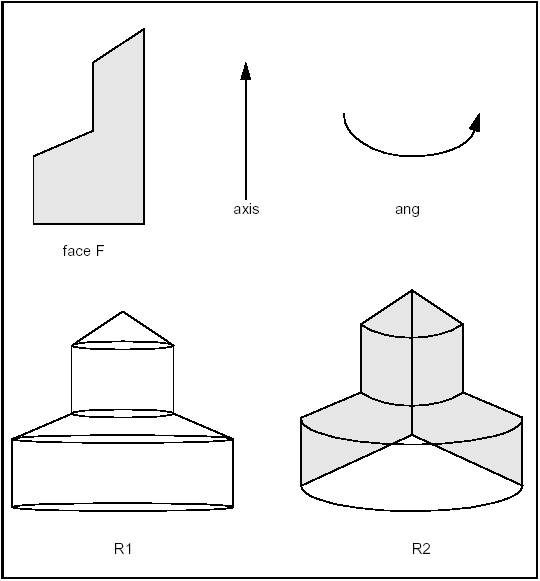Full and partial rotation

# Boolean Operations

Boolean operations are used to create new shapes from the combinations of two shapes.

Operation Result
Fuse all points in S1 or S2
Common all points in S1 and S2
Cut S1 by S2all points in S1 and not in S2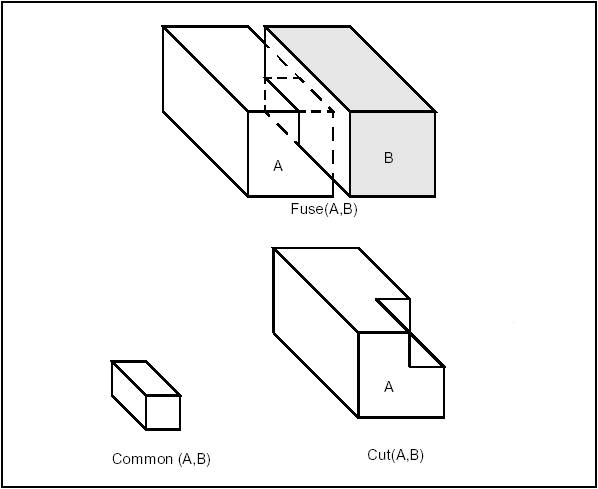Boolean Operations

From the viewpoint of Topology these are topological operations followed by blending (putting fillets onto edges created after the topological operation).

Topological operations are the most convenient way to create real industrial parts. As most industrial parts consist of several simple elements such as gear wheels, arms, holes, ribs, tubes and pipes. It is usually easy to create those elements separately and then to combine them by Boolean operations in the whole final part.

See Boolean Operations for detailed documentation.

## Input and Result Arguments

Boolean Operations have the following types of the arguments and produce the following results:

• For arguments having the same shape type (e.g. SOLID / SOLID) the type of the resulting shape will be a COMPOUND, containing shapes of this type;
• For arguments having different shape types (e.g. SHELL / SOLID) the type of the resulting shape will be a COMPOUND, containing shapes of the type that is the same as that of the low type of the argument. Example: For SHELL/SOLID the result is a COMPOUND of SHELLs.
• For arguments with different shape types some of Boolean Operations can not be done using the default implementation, because of a non-manifold type of the result. Example: the FUSE operation for SHELL and SOLID can not be done, but the CUT operation can be done, where SHELL is the object and SOLID is the tool.
• It is possible to perform Boolean Operations on arguments of the COMPOUND shape type. In this case each compound must not be heterogeneous, i.e. it must contain equidimensional shapes (EDGEs or/and WIREs, FACEs or/and SHELLs, SOLIDs). SOLIDs inside the COMPOUND must not contact (intersect or touch) each other. The same condition should be respected for SHELLs or FACEs, WIREs or EDGEs.
• Boolean Operations for COMPSOLID type of shape are not supported.

## Implementation

BRepAlgoAPI_BooleanOperation class is the deferred root class for Boolean operations.

#### Fuse

BRepAlgoAPI_Fuse performs the Fuse operation.

TopoDS_Shape A = ..., B = ...;
TopoDS_Shape S = BRepAlgoAPI_Fuse(A,B);

#### Common

BRepAlgoAPI_Common performs the Common operation.

TopoDS_Shape A = ..., B = ...;
TopoDS_Shape S = BRepAlgoAPI_Common(A,B);

#### Cut

BRepAlgoAPI_Cut performs the Cut operation.

TopoDS_Shape A = ..., B = ...;
TopoDS_Shape S = BRepAlgoAPI_Cut(A,B);

#### Section

BRepAlgoAPI_Section performs the section, described as a TopoDS_Compound made of TopoDS_Edge.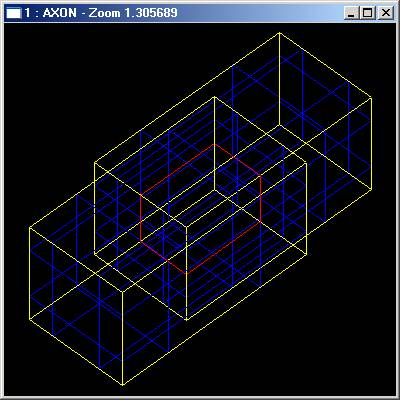Section operation
TopoDS_Shape A = ..., TopoDS_ShapeB = ...;
TopoDS_Shape S = BRepAlgoAPI_Section(A,B);

# Fillets and Chamfers

This library provides algorithms to make fillets and chamfers on shape edges. The following cases are addressed:

• Corners and apexes with different radii;
• Corners and apexes with different concavity.

If there is a concavity, both surfaces that need to be extended and those, which do not, are processed.

## Fillet on shape

A fillet is a smooth face replacing a sharp edge.

BRepFilletAPI_MakeFillet class allows filleting a shape.

To produce a fillet, it is necessary to define the filleted shape at the construction of the class and add fillet descriptions using the Add method.

A fillet description contains an edge and a radius. The edge must be shared by two faces. The fillet is automatically extended to all edges in a smooth continuity with the original edge. It is not an error to add a fillet twice, the last description holds.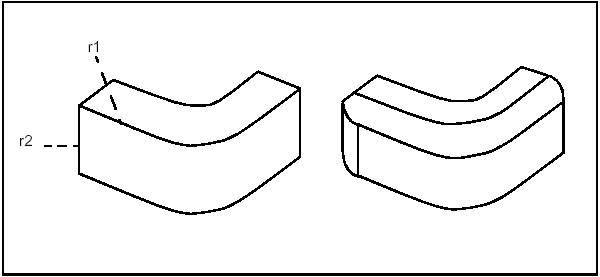Filleting two edges using radii r1 and r2.

In the following example a filleted box with dimensions a,b,c and radius r is created.

#include <TopoDS_Shape.hxx>
#include <TopoDS.hxx>
#include <BRepPrimAPI_MakeBox.hxx>
#include <TopoDS_Solid.hxx>
#include <BRepFilletAPI_MakeFillet.hxx>
#include <TopExp_Explorer.hxx>
TopoDS_Shape FilletedBox(const Standard_Real a,
const Standard_Real b,
const Standard_Real c,
const Standard_Real r)
{
TopoDS_Solid Box = BRepPrimAPI_MakeBox(a,b,c);
BRepFilletAPI_MakeFillet MF(Box);
// add all the edges to fillet
TopExp_Explorer ex(Box,TopAbs_EDGE);
while (ex.More())
{
ex.Next();
}
return MF.Shape();
}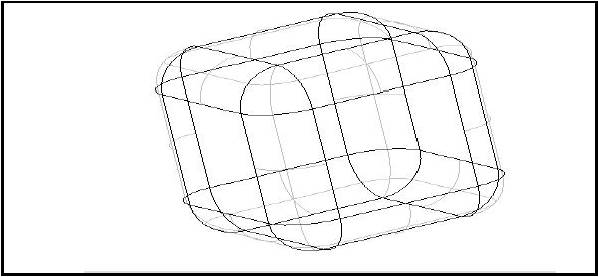void CSampleTopologicalOperationsDoc::OnEvolvedblend1()
{
TopoDS_Shape theBox = BRepPrimAPI_MakeBox(200,200,200);
BRepFilletAPI_MakeFillet Rake(theBox);
ChFi3d_FilletShape FSh = ChFi3d_Rational;
Rake.SetFilletShape(FSh);
TopExp_Explorer ex(theBox,TopAbs_EDGE);
TopoDS_Shape evolvedBox = Rake.Shape();
}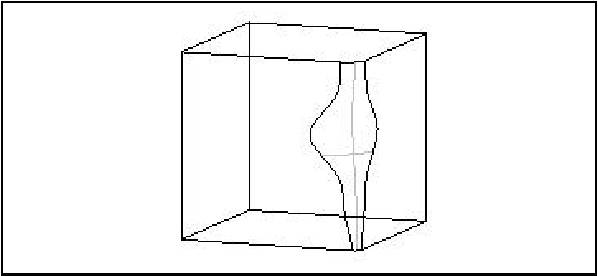## Chamfer

A chamfer is a rectilinear edge replacing a sharp vertex of the face.

The use of BRepFilletAPI_MakeChamfer class is similar to the use of BRepFilletAPI_MakeFillet, except for the following:

• The surfaces created are ruled and not smooth.
• The Add syntax for selecting edges requires one or two distances, one edge and one face (contiguous to the edge).
Add(d1, d2, E, F) with d1 on the face F.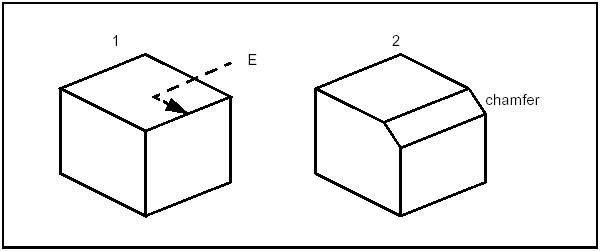Chamfer

## Fillet on a planar face

BRepFilletAPI_MakeFillet2d class allows constructing fillets and chamfers on planar faces. To create a fillet on planar face: define it, indicate, which vertex is to be deleted, and give the fillet radius with AddFillet method.

A chamfer can be calculated with AddChamfer method. It can be described by

• two edges and two distances
• one edge, one vertex, one distance and one angle. Fillets and chamfers are calculated when addition is complete.

If face F2 is created by 2D fillet and chamfer builder from face F1, the builder can be rebuilt (the builder recovers the status it had before deletion). To do so, use the following syntax:

BRepFilletAPI_MakeFillet2d builder;
builder.Init(F1,F2);

## Planar Fillet

#include “BRepPrimAPI_MakeBox.hxx”
#include “TopoDS_Shape.hxx”
#include “TopExp_Explorer.hxx”
#include “BRepFilletAPI_MakeFillet2d.hxx”
#include “TopoDS.hxx”
#include “TopoDS_Solid.hxx”
TopoDS_Shape FilletFace(const Standard_Real a,
const Standard_Real b,
const Standard_Real c,
const Standard_Real r)
{
TopoDS_Solid Box = BRepPrimAPI_MakeBox (a,b,c);
TopExp_Explorer ex1(Box,TopAbs_FACE);
const TopoDS_Face& F = TopoDS::Face(ex1.Current());
BRepFilletAPI_MakeFillet2d MF(F);
TopExp_Explorer ex2(F, TopAbs_VERTEX);
while (ex2.More())
{
ex2.Next();
}
// while...
return MF.Shape();
}

# Offsets, Drafts, Pipes and Evolved shapes

These classes provide the following services:

• Creation of offset shapes and their variants such as:
• Hollowing;
• Shelling;
• Lofting;
• Creation of tapered shapes using draft angles;
• Creation of sweeps.

## Offset computation

Offset computation can be performed using BRepOffsetAPI_MakeOffsetShape. This class provides API to the two different offset algorithms:

Offset algorithm based on computation of the analytical continuation. Meaning of the parameters can be found in BRepOffsetAPI_MakeOffsetShape::PerformByJoin method description. The list below demonstrates principal scheme of this algorithm:

• At the first step, the offsets are computed.
• After this, the analytical continuations are computed for each offset.
• Pairwise intersection is computed according to the original topological information (sharing, number of neighbors, etc.).
• The offset shape is assembled.

The second algorithm is based on the fact that the offset computation for a single face without continuation can always be built. The list below shows simple offset algorithm:

• Each surface is mapped to its geometric offset surface.
• For each edge, pcurves are mapped to the same pcurves on offset surfaces.
• For each edge, 3d curve is constructed by re-approximation of pcurve on the first offset face.
• Position of each vertex in a result shell is computed as average point of all ends of edges sharing that vertex.
• Tolerances are updated according to the resulting geometry. The possible drawback of the simple algorithm is that it leads, in general case, to tolerance increasing. The tolerances have to grow in order to cover the gaps between the neighbor faces in the output. It should be noted that the actual tolerance growth depends on the offset distance and the quality of joints between the input faces. Anyway the good input shell (smooth connections between adjacent faces) will lead to good result.

The snippets below show usage examples:

BRepOffsetAPI_MakeOffsetShape OffsetMaker1;
// Computes offset shape using analytical continuation mechanism.
OffsetMaker1.PerformByJoin(Shape, OffsetValue, Tolerance);
if (OffsetMaker1.IsDone())
NewShape = OffsetMaker1.Shape();
BRepOffsetAPI_MakeOffsetShape OffsetMaker2;
// Computes offset shape using simple algorithm.
OffsetMaker2.PerformBySimple(Shape, OffsetValue);
if (OffsetMaker2.IsDone())
NewShape = OffsetMaker2.Shape();

## Shelling

Shelling is used to offset given faces of a solid by a specific value. It rounds or intersects adjacent faces along its edges depending on the convexity of the edge. The MakeThickSolidByJoin method of the BRepOffsetAPI_MakeThickSolid takes the solid, the list of faces to remove and an offset value as input.

TopoDS_Solid SolidInitial = ...;
Standard_Real Of = ...;
TopTools_ListOfShape LCF;
TopoDS_Shape Result;
Standard_Real Tol = Precision::Confusion();
for (Standard_Integer i = 1 ;i <= n; i++) {
TopoDS_Face SF = ...; // a face from SolidInitial
LCF.Append(SF);
}
BRepOffsetAPI_MakeThickSolid SolidMaker;
SolidMaker.MakeThickSolidByJoin(SolidInitial,
LCF,
Of,
Tol);
if (SolidMaker.IsDone())
Result = SolidMaker.Shape();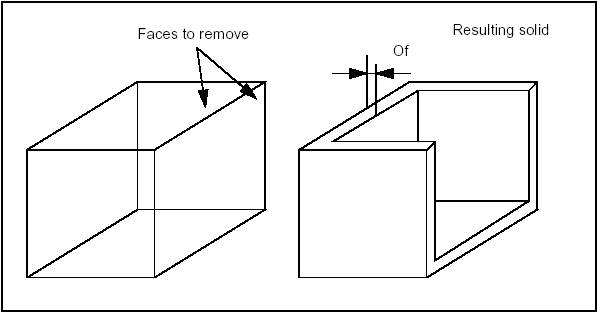Shelling

Also it is possible to create solid between shell, offset shell. This functionality can be called using BRepOffsetAPI_MakeThickSolid::MakeThickSolidBySimple method. The code below shows usage example:

BRepOffsetAPI_MakeThickSolid SolidMaker;
SolidMaker.MakeThickSolidBySimple(Shell, OffsetValue);
if (myDone.IsDone())
Solid = SolidMaker.Shape();

## Draft Angle

BRepOffsetAPI_DraftAngle class allows modifying a shape by applying draft angles to its planar, cylindrical and conical faces.

The class is created or initialized from a shape, then faces to be modified are added; for each face, three arguments are used:

• Direction: the direction with which the draft angle is measured
• Angle: value of the angle
• Neutral plane: intersection between the face and the neutral plane is invariant.

The following code places a draft angle on several faces of a shape; the same direction, angle and neutral plane are used for each face:

TopoDS_Shape myShape = ...
// The original shape
TopTools_ListOfShape ListOfFace;
// Creation of the list of faces to be modified
...
gp_Dir Direc(0.,0.,1.);
// Z direction
Standard_Real Angle = 5.*PI/180.;
// 5 degree angle
gp_Pln Neutral(gp_Pnt(0.,0.,5.), Direc);
// Neutral plane Z=5
BRepOffsetAPI_DraftAngle theDraft(myShape);
TopTools_ListIteratorOfListOfShape itl;
for (itl.Initialize(ListOfFace); itl.More(); itl.Next()) {
// An error has occurred. The faulty face is given by // ProblematicShape
break;
}
}
// An error has occurred
TopoDS_Face guilty = theDraft.ProblematicShape();
...
}
theDraft.Build();
if (!theDraft.IsDone()) {
// Problem encountered during reconstruction
...
}
else {
TopoDS_Shape myResult = theDraft.Shape();
...
}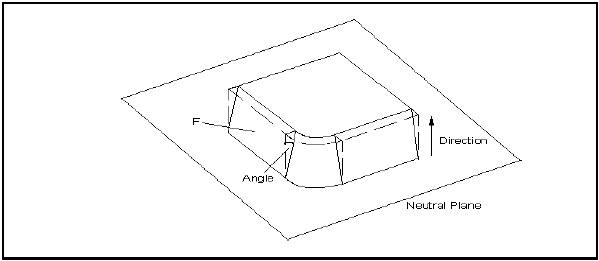DraftAngle

## Pipe Constructor

BRepOffsetAPI_MakePipe class allows creating a pipe from a Spine, which is a Wire and a Profile which is a Shape. This implementation is limited to spines with smooth transitions, sharp transitions are precessed by BRepOffsetAPI_MakePipeShell. To be more precise the continuity must be G1, which means that the tangent must have the same direction, though not necessarily the same magnitude, at neighboring edges.

The angle between the spine and the profile is preserved throughout the pipe.

TopoDS_Wire Spine = ...;
TopoDS_Shape Profile = ...;
TopoDS_Shape Pipe = BRepOffsetAPI_MakePipe(Spine,Profile);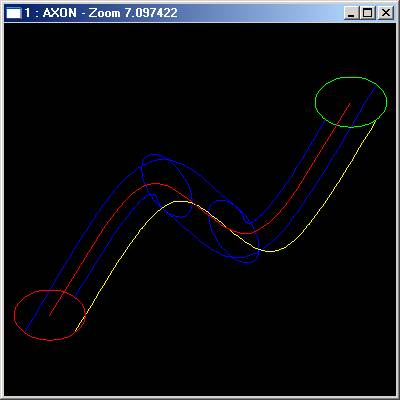Example of a Pipe

## Evolved Solid

BRepOffsetAPI_MakeEvolved class allows creating an evolved solid from a Spine (planar face or wire) and a profile (wire).

The evolved solid is an unlooped sweep generated by the spine and the profile.

The evolved solid is created by sweeping the profile’s reference axes on the spine. The origin of the axes moves to the spine, the X axis and the local tangent coincide and the Z axis is normal to the face.

The reference axes of the profile can be defined following two distinct modes:

• The reference axes of the profile are the origin axes.
• The references axes of the profile are calculated as follows:
• the origin is given by the point on the spine which is the closest to the profile
• the X axis is given by the tangent to the spine at the point defined above
• the Z axis is the normal to the plane which contains the spine.
TopoDS_Face Spine = ...;
TopoDS_Wire Profile = ...;
TopoDS_Shape Evol =
BRepOffsetAPI_MakeEvolved(Spine,Profile);

# Sewing

## Introduction

Sewing allows creation of connected topology (shells and wires) from a set of separate topological elements (faces and edges). For example, Sewing can be used to create of shell from a compound of separate faces.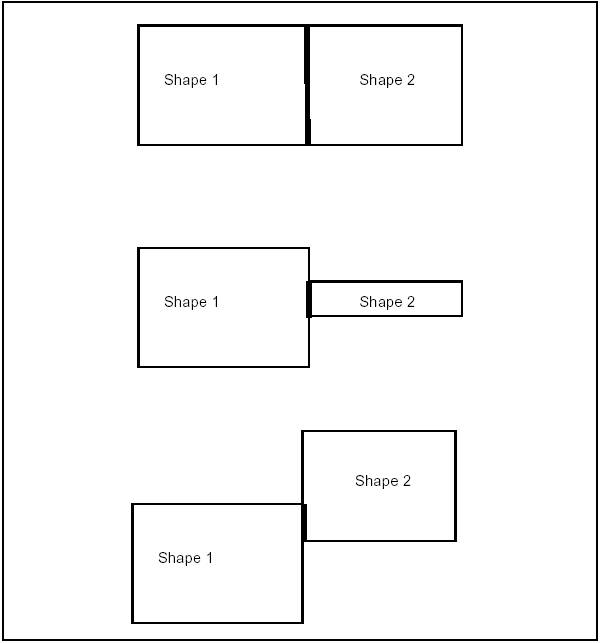Shapes with partially shared edges

It is important to distinguish between sewing and other procedures, which modify the geometry, such as filling holes or gaps, gluing, bending curves and surfaces, etc.

Sewing does not change geometrical representation of the shapes. Sewing applies to topological elements (faces, edges) which are not connected but can be connected because they are geometrically coincident : it adds the information about topological connectivity. Already connected elements are left untouched in case of manifold sewing.

Let us define several terms:

• Floating edges do not belong to any face;
• Free boundaries belong to one face only;
• Shared edges belong to several faces, (i.e. two faces in a manifold topology).
• Sewn faces should have edges shared with each other.
• Sewn edges should have vertices shared with each other.

## Sewing Algorithm

The sewing algorithm is one of the basic algorithms used for shape processing, therefore its quality is very important.

Sewing algorithm is implemented in the class BRepBuilder_Sewing. This class provides the following methods:

• setting customization parameters, such as special operation modes, tolerances and output results;
• applying analysis methods that can be used to obtain connectivity data required by external algorithms;
• sewing of the loaded shapes.

Sewing supports working mode with big value tolerance. It is not necessary to repeat sewing step by step while smoothly increasing tolerance.

It is also possible to sew edges to wire and to sew locally separate faces and edges from a shape.

The Sewing algorithm can be subdivided into several independent stages, some of which can be turned on or off using Boolean or other flags.

In brief, the algorithm should find a set of merge candidates for each free boundary, filter them according to certain criteria, and finally merge the found candidates and build the resulting sewn shape.

Each stage of the algorithm or the whole algorithm can be adjusted with the following parameters:

• Working tolerance defines the maximal distance between topological elements which can be sewn. It is not ultimate that such elements will be actually sewn as many other criteria are applied to make the final decision.
• Minimal tolerance defines the size of the smallest element (edge) in the resulting shape. It is declared that no edges with size less than this value are created after sewing. If encountered, such topology becomes degenerated.
• Non-manifold mode enables sewing of non-manifold topology.

#### Example

To connect a set of n contiguous but independent faces, do the following:

BRepBuilderAPI_Sewing Sew;
...
Sew.Perform();
TopoDS_Shape result= Sew.SewedShape();

If all faces have been sewn correctly, the result is a shell. Otherwise, it is a compound. After a successful sewing operation all faces have a coherent orientation.

## Tolerance Management

To produce a closed shell, Sewing allows specifying the value of working tolerance, exceeding the size of small faces belonging to the shape.

However, if we produce an open shell, it is possible to get incorrect sewing results if the value of working tolerance is too large (i.e. it exceeds the size of faces lying on an open boundary).

The following recommendations can be proposed for tuning-up the sewing process:

• Use as small working tolerance as possible. This will reduce the sewing time and, consequently, the number of incorrectly sewn edges for shells with free boundaries.
• Use as large minimal tolerance as possible. This will reduce the number of small geometry in the shape, both original and appearing after cutting.
• If it is expected to obtain a shell with holes (free boundaries) as a result of sewing, the working tolerance should be set to a value not greater than the size of the smallest element (edge) or smallest distance between elements of such free boundary. Otherwise the free boundary may be sewn only partially.
• It should be mentioned that the Sewing algorithm is unable to understand which small (less than working tolerance) free boundary should be kept and which should be sewn.

## Manifold and Non-manifold Sewing

To create one or several shells from a set of faces, sewing merges edges, which belong to different faces or one closed face.

Face sewing supports manifold and non manifold modes. Manifold mode can produce only a manifold shell. Sewing should be used in the non manifold mode to create non manifold shells.

Manifold sewing of faces merges only two nearest edges belonging to different faces or one closed face with each other. Non manifold sewing of faces merges all edges at a distance less than the specified tolerance.

For a complex topology it is advisable to apply first the manifold sewing and then the non manifold sewing a minimum possible working tolerance. However, this is not necessary for a easy topology.

Giving a large tolerance value to non manifold sewing will cause a lot of incorrectness since all nearby geometry will be sewn.

## Local Sewing

If a shape still has some non-sewn faces or edges after sewing, it is possible to use local sewing with a greater tolerance.

Local sewing is especially good for open shells. It allows sewing an unwanted hole in one part of the shape and keeping a required hole, which is smaller than the working tolerance specified for the local sewing in the other part of the shape. Local sewing is much faster than sewing on the whole shape.

All preexisting connections of the whole shape are kept after local sewing.

For example, if you want to sew two open shells having coincided free edges using local sewing, it is necessary to create a compound from two shells then load the full compound using method BRepBuilderAPI_Sewing::Load(). After that it is necessary to add local sub-shapes, which should be sewn using method BRepBuilderAPI_Sewing::Add(). The result of sewing can be obtained using method BRepBuilderAPI_Sewing::SewedShape().

See the example:

//initial sewn shapes
TopoDS_Shape aS1, aS2; // these shapes are expected to be well sewn shells
TopoDS_Shape aComp;
BRep_Builder aB;
aB.MakeCompound(aComp);
................................
//sub shapes which should be locally sewed
//performing sewing
aSewing.Perform();
//result shape
TopoDS_Shape aRes = aSewing.SewedShape();

# Features

This library contained in BRepFeat package is necessary for creation and manipulation of form and mechanical features that go beyond the classical boundary representation of shapes. In that sense, BRepFeat is an extension of BRepBuilderAPI package.

## Form Features

The form features are depressions or protrusions including the following types:

• Cylinder;
• Draft Prism;
• Prism;
• Revolved feature;
• Pipe.

Depending on whether you wish to make a depression or a protrusion, you can choose either to remove matter (Boolean cut: Fuse equal to 0) or to add it (Boolean fusion: Fuse equal to 1).

The semantics of form feature creation is based on the construction of shapes:

• for a certain length in a certain direction;
• up to the limiting face;
• from the limiting face at a height;
• above and/or below a plane.

The shape defining the construction of a feature can be either a supporting edge or a concerned area of a face.

In case of supporting edge, this contour can be attached to a face of the basis shape by binding. When the contour is bound to this face, the information that the contour will slide on the face becomes available to the relevant class methods. In case of the concerned area of a face, you can, for example, cut it out and move it at a different height, which defines the limiting face of a protrusion or depression.

Topological definition with local operations of this sort makes calculations simpler and faster than a global operation. The latter would entail a second phase of removing unwanted matter to get the same result.

The Form from BRepFeat package is a deferred class used as a root for form features. It inherits MakeShape from BRepBuilderAPI and provides implementation of methods keep track of all sub-shapes.

### Prism

The class BRepFeat_MakePrism is used to build a prism interacting with a shape. It is created or initialized from

• a shape (the basic shape),
• the base of the prism,
• a face (the face of sketch on which the base has been defined and used to determine whether the base has been defined on the basic shape or not),
• a direction,
• a Boolean indicating the type of operation (fusion=protrusion or cut=depression) on the basic shape,
• another Boolean indicating if the self-intersections have to be found (not used in every case).

There are six Perform methods:

Method Description
Perform(Height) The resulting prism is of the given length.
Perform(Until) The prism is defined between the position of the base and the given face.
Perform(From, Until) The prism is defined between the two faces From and Until.
PerformUntilEnd() The prism is semi-infinite, limited by the actual position of the base.
PerformFromEnd(Until) The prism is semi-infinite, limited by the face Until.
PerformThruAll() The prism is infinite. In the case of a depression, the result is similar to a cut with an infinite prism. In the case of a protrusion, infinite parts are not kept in the result.

Note that Add method can be used before Perform methods to indicate that a face generated by an edge slides onto a face of the base shape.

In the following sequence, a protrusion is performed, i.e. a face of the shape is changed into a prism.

TopoDS_Shape Sbase = ...; // an initial shape
TopoDS_Face Fbase = ....; // a base of prism
gp_Dir Extrusion (.,.,.);
// An empty face is given as the sketch face
BRepFeat_MakePrism thePrism(Sbase, Fbase, TopoDS_Face(), Extrusion, Standard_True, Standard_True);
thePrism, Perform(100.);
if (thePrism.IsDone()) {
TopoDS_Shape theResult = thePrism;
...
}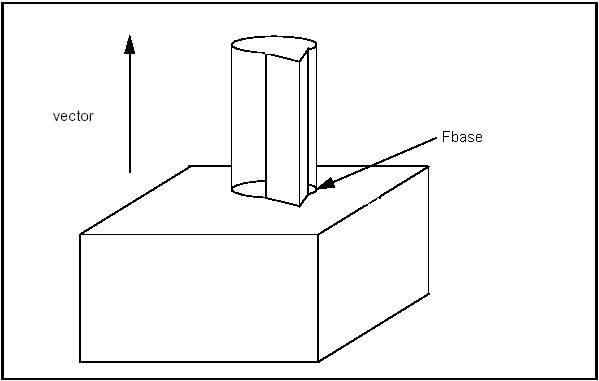Fusion with MakePrism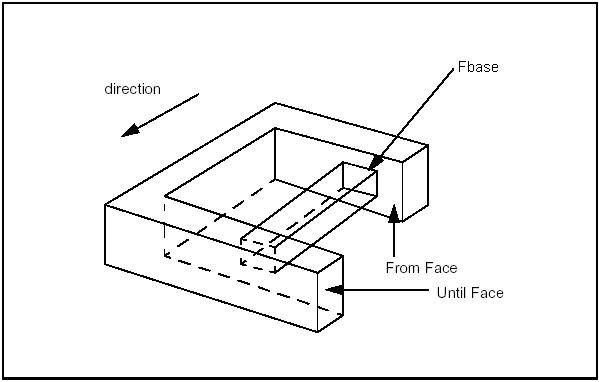Creating a prism between two faces with Perform()

### Draft Prism

The class BRepFeat_MakeDPrism is used to build draft prism topologies interacting with a basis shape. These can be depressions or protrusions. A class object is created or initialized from:

• a shape (basic shape),
• the base of the prism,
• a face (face of sketch on which the base has been defined and used to determine whether the base has been defined on the basic shape or not),
• an angle,
• a Boolean indicating the type of operation (fusion=protrusion or cut=depression) on the basic shape,
• another Boolean indicating if self-intersections have to be found (not used in every case).

Evidently the input data for MakeDPrism are the same as for MakePrism except for a new parameter Angle and a missing parameter Direction: the direction of the prism generation is determined automatically as the normal to the base of the prism. The semantics of draft prism feature creation is based on the construction of shapes:

• along a length
• up to a limiting face
• from a limiting face to a height.

The shape defining construction of the draft prism feature can be either the supporting edge or the concerned area of a face.

In case of the supporting edge, this contour can be attached to a face of the basis shape by binding. When the contour is bound to this face, the information that the contour will slide on the face becomes available to the relevant class methods. In case of the concerned area of a face, it is possible to cut it out and move it to a different height, which will define the limiting face of a protrusion or depression direction .

The Perform methods are the same as for MakePrism.

TopoDS_Shape S = BRepPrimAPI_MakeBox(400.,250.,300.);
TopExp_Explorer Ex;
Ex.Init(S,TopAbs_FACE);
Ex.Next();
Ex.Next();
Ex.Next();
Ex.Next();
Ex.Next();
TopoDS_Face F = TopoDS::Face(Ex.Current());
Handle(Geom_Surface) surf = BRep_Tool::Surface(F);
gp_Circ2d
c(gp_Ax2d(gp_Pnt2d(200.,130.),gp_Dir2d(1.,0.)),50.);
BRepBuilderAPI_MakeWire MW;
Handle(Geom2d_Curve) aline = new Geom2d_Circle(c);
BRepBuilderAPI_MakeFace MKF;
MKF.Init(surf,Standard_False);
TopoDS_Face FP = MKF.Face();
BRepLib::BuildCurves3d(FP);
BRepFeat_MakeDPrism MKDP (S,FP,F,10*PI180,Standard_True,
Standard_True);
MKDP.Perform(200);
TopoDS_Shape res1 = MKDP.Shape();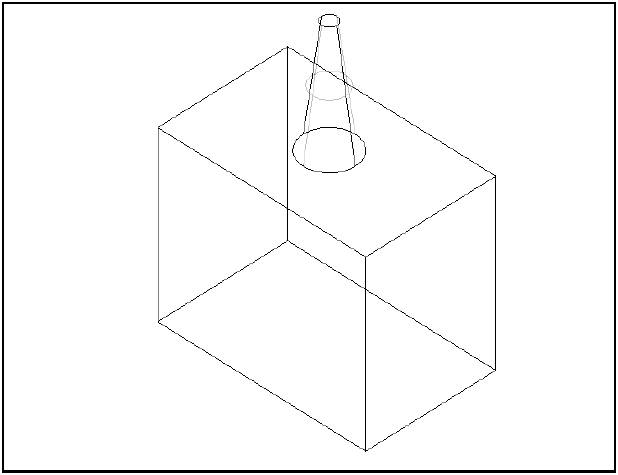A tapered prism

### Revolution

The class BRepFeat_MakeRevol is used to build a revolution interacting with a shape. It is created or initialized from:

• a shape (the basic shape,)
• the base of the revolution,
• a face (the face of sketch on which the base has been defined and used to determine whether the base has been defined on the basic shape or not),
• an axis of revolution,
• a boolean indicating the type of operation (fusion=protrusion or cut=depression) on the basic shape,
• another boolean indicating whether the self-intersections have to be found (not used in every case).

There are four Perform methods:

Method Description
Perform(Angle) The resulting revolution is of the given magnitude.
Perform(Until) The revolution is defined between the actual position of the base and the given face.
Perform(From, Until) The revolution is defined between the two faces, From and Until.
PerformThruAll() The result is similar to Perform(2*PI).

Note that Add method can be used before Perform methods to indicate that a face generated by an edge slides onto a face of the base shape.

In the following sequence, a face is revolved and the revolution is limited by a face of the base shape.

TopoDS_Shape Sbase = ...; // an initial shape
TopoDS_Face Frevol = ....; // a base of prism
TopoDS_Face FUntil = ....; // face limiting the revol
gp_Dir RevolDir (.,.,.);
gp_Ax1 RevolAx(gp_Pnt(.,.,.), RevolDir);
// An empty face is given as the sketch face
BRepFeat_MakeRevol theRevol(Sbase, Frevol, TopoDS_Face(), RevolAx, Standard_True, Standard_True);
theRevol.Perform(FUntil);
if (theRevol.IsDone()) {
TopoDS_Shape theResult = theRevol;
...
}

### Pipe

The class BRepFeat_MakePipe constructs compound shapes with pipe features: depressions or protrusions. A class object is created or initialized from:

• a shape (basic shape),
• a base face (profile of the pipe)
• a face (face of sketch on which the base has been defined and used to determine whether the base has been defined on the basic shape or not),
• a spine wire
• a Boolean indicating the type of operation (fusion=protrusion or cut=depression) on the basic shape,
• another Boolean indicating if self-intersections have to be found (not used in every case).

There are three Perform methods:

Method Description
Perform() The pipe is defined along the entire path (spine wire)
Perform(Until) The pipe is defined along the path until a given face
Perform(From, Until) The pipe is defined between the two faces From and Until

Let us have a look at the example:

TopoDS_Shape S = BRepPrimAPI_MakeBox(400.,250.,300.);
TopExp_Explorer Ex;
Ex.Init(S,TopAbs_FACE);
Ex.Next();
Ex.Next();
TopoDS_Face F1 = TopoDS::Face(Ex.Current());
Handle(Geom_Surface) surf = BRep_Tool::Surface(F1);
BRepBuilderAPI_MakeWire MW1;
gp_Pnt2d p1,p2;
p1 = gp_Pnt2d(100.,100.);
p2 = gp_Pnt2d(200.,100.);
Handle(Geom2d_Line) aline = GCE2d_MakeLine(p1,p2).Value();
p1 = p2;
p2 = gp_Pnt2d(150.,200.);
aline = GCE2d_MakeLine(p1,p2).Value();
p1 = p2;
p2 = gp_Pnt2d(100.,100.);
aline = GCE2d_MakeLine(p1,p2).Value();
BRepBuilderAPI_MakeFace MKF1;
MKF1.Init(surf,Standard_False);
TopoDS_Face FP = MKF1.Face();
BRepLib::BuildCurves3d(FP);
TColgp_Array1OfPnt CurvePoles(1,3);
gp_Pnt pt = gp_Pnt(150.,0.,150.);
CurvePoles(1) = pt;
pt = gp_Pnt(200.,100.,150.);
CurvePoles(2) = pt;
pt = gp_Pnt(150.,200.,150.);
CurvePoles(3) = pt;
Handle(Geom_BezierCurve) curve = new Geom_BezierCurve
(CurvePoles);
TopoDS_Edge E = BRepBuilderAPI_MakeEdge(curve);
TopoDS_Wire W = BRepBuilderAPI_MakeWire(E);
BRepFeat_MakePipe MKPipe (S,FP,F1,W,Standard_False,
Standard_True);
MKPipe.Perform();
TopoDS_Shape res1 = MKPipe.Shape();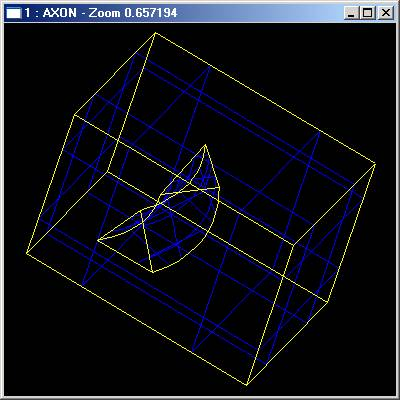Pipe depression

## Mechanical Features

Mechanical features include ribs, protrusions and grooves (or slots), depressions along planar (linear) surfaces or revolution surfaces.

The semantics of mechanical features is built around giving thickness to a contour. This thickness can either be symmetrical – on one side of the contour – or dissymmetrical – on both sides. As in the semantics of form features, the thickness is defined by construction of shapes in specific contexts.

The development contexts differ, however, in the case of mechanical features. Here they include extrusion:

• to a limiting face of the basis shape;
• to or from a limiting plane;
• to a height.

A class object is created or initialized from

• a shape (basic shape);
• a wire (base of rib or groove);
• a plane (plane of the wire);
• direction1 (a vector along which thickness will be built up);
• direction2 (vector opposite to the previous one along which thickness will be built up, may be null);
• a Boolean indicating the type of operation (fusion=rib or cut=groove) on the basic shape;
• another Boolean indicating if self-intersections have to be found (not used in every case).

### Linear Form

Linear form is implemented in MakeLinearForm class, which creates a rib or a groove along a planar surface. There is one Perform() method, which performs a prism from the wire along the direction1 and direction2 interacting with base shape Sbase. The height of the prism is Magnitude(Direction1)+Magnitude(direction2).

BRepBuilderAPI_MakeWire mkw;
gp_Pnt p1 = gp_Pnt(0.,0.,0.);
gp_Pnt p2 = gp_Pnt(200.,0.,0.);
p1 = p2;
p2 = gp_Pnt(200.,0.,50.);
p1 = p2;
p2 = gp_Pnt(50.,0.,50.);
p1 = p2;
p2 = gp_Pnt(50.,0.,200.);
p1 = p2;
p2 = gp_Pnt(0.,0.,200.);
p1 = p2;
TopoDS_Shape S = BRepBuilderAPI_MakePrism(BRepBuilderAPI_MakeFace
(mkw.Wire()),gp_Vec(gp_Pnt(0.,0.,0.),gp_P
nt(0.,100.,0.)));
TopoDS_Wire W = BRepBuilderAPI_MakeWire(BRepBuilderAPI_MakeEdge(gp_Pnt
(50.,45.,100.),
gp_Pnt(100.,45.,50.)));
Handle(Geom_Plane) aplane =
new Geom_Plane(gp_Pnt(0.,45.,0.), gp_Vec(0.,1.,0.));
BRepFeat_MakeLinearForm aform(S, W, aplane, gp_Dir
(0.,5.,0.), gp_Dir(0.,-3.,0.), 1, Standard_True);
aform.Perform();
TopoDS_Shape res = aform.Shape();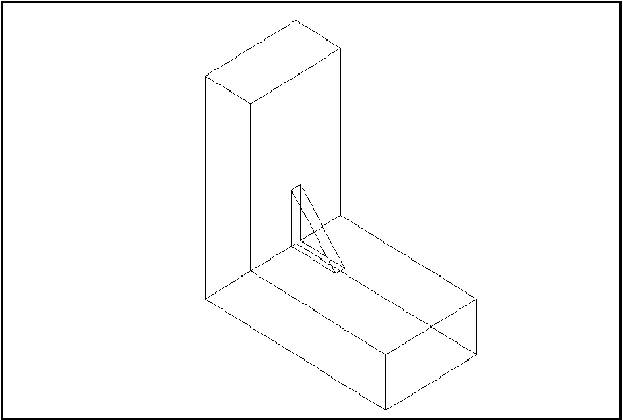Creating a rib

### Gluer

The class BRepFeat_Gluer allows gluing two solids along faces. The contact faces of the glued shape must not have parts outside the contact faces of the basic shape. Upon completion the algorithm gives the glued shape with cut out parts of faces inside the shape.

The class is created or initialized from two shapes: the “glued” shape and the basic shape (on which the other shape is glued). Two Bind methods are used to bind a face of the glued shape to a face of the basic shape and an edge of the glued shape to an edge of the basic shape.

Note that every face and edge has to be bounded, if two edges of two glued faces are coincident they must be explicitly bounded.

TopoDS_Shape Sbase = ...; // the basic shape
TopoDS_Shape Sglued = ...; // the glued shape
TopTools_ListOfShape Lfbase;
TopTools_ListOfShape Lfglued;
// Determination of the glued faces
...
BRepFeat_Gluer theGlue(Sglue, Sbase);
TopTools_ListIteratorOfListOfShape itlb(Lfbase);
TopTools_ListIteratorOfListOfShape itlg(Lfglued);
for (; itlb.More(); itlb.Next(), itlg(Next()) {
const TopoDS_Face& f1 = TopoDS::Face(itlg.Value());
const TopoDS_Face& f2 = TopoDS::Face(itlb.Value());
theGlue.Bind(f1,f2);
// for example, use the class FindEdges from LocOpe to
// determine coincident edges
LocOpe_FindEdge fined(f1,f2);
for (fined.InitIterator(); fined.More(); fined.Next()) {
theGlue.Bind(fined.EdgeFrom(),fined.EdgeTo());
}
}
theGlue.Build();
if (theGlue.IsDone() {
TopoDS_Shape theResult = theGlue;
...
}

### Split Shape

The class BRepFeat_SplitShape is used to split faces of a shape into wires or edges. The shape containing the new entities is rebuilt, sharing the unmodified ones.

The class is created or initialized from a shape (the basic shape). Three Add methods are available:

• Add(Wire, Face) – adds a new wire on a face of the basic shape.
• Add(Edge, Face) – adds a new edge on a face of the basic shape.
• Add(EdgeNew, EdgeOld) – adds a new edge on an existing one (the old edge must contain the new edge).

Note The added wires and edges must define closed wires on faces or wires located between two existing edges. Existing edges must not be intersected.

TopoDS_Shape Sbase = ...; // basic shape
TopoDS_Face Fsplit = ...; // face of Sbase
TopoDS_Wire Wsplit = ...; // new wire contained in Fsplit
BRepFeat_SplitShape Spls(Sbase);
TopoDS_Shape theResult = Spls;
...

# Hidden Line Removal

To provide the precision required in industrial design, drawings need to offer the possibility of removing lines, which are hidden in a given projection.

For this the Hidden Line Removal component provides two algorithms: HLRBRep_Algo and HLRBRep_PolyAlgo.

These algorithms are based on the principle of comparing each edge of the shape to be visualized with each of its faces, and calculating the visible and the hidden parts of each edge. Note that these are not the algorithms used in generating shading, which calculate the visible and hidden parts of each face in a shape to be visualized by comparing each face in the shape with every other face in the same shape. These algorithms operate on a shape and remove or indicate edges hidden by faces. For a given projection, they calculate a set of lines characteristic of the object being represented. They are also used in conjunction with extraction utilities, which reconstruct a new, simplified shape from a selection of the results of the calculation. This new shape is made up of edges, which represent the shape visualized in the projection.

HLRBRep_Algo allows working with the shape itself, whereas HLRBRep_PolyAlgo works with a polyhedral simplification of the shape. When you use HLRBRep_Algo, you obtain an exact result, whereas, when you use HLRBRep_PolyAlgo, you reduce the computation time, but obtain polygonal segments.

No smoothing algorithm is provided. Consequently, a polyhedron will be treated as such and the algorithms will give the results in form of line segments conforming to the mathematical definition of the polyhedron. This is always the case with HLRBRep_PolyAlgo.

HLRBRep_Algo and HLRBRep_PolyAlgo can deal with any kind of object, for example, assemblies of volumes, surfaces, and lines, as long as there are no unfinished objects or points within it.

However, there some restrictions in HLR use:

• Points are not processed;
• Infinite faces or lines are not processed.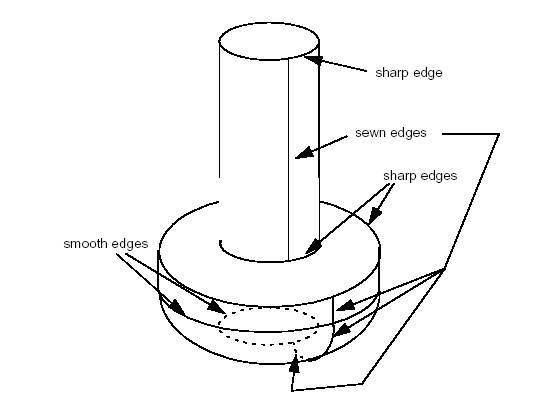,"Sharp, smooth and sewn edges in a simple screw shape",320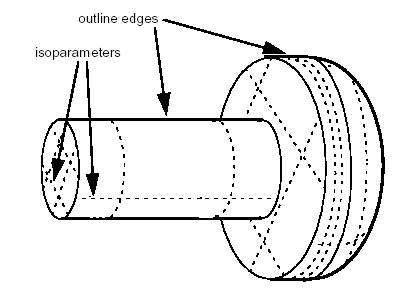Outline edges and isoparameters in the same shape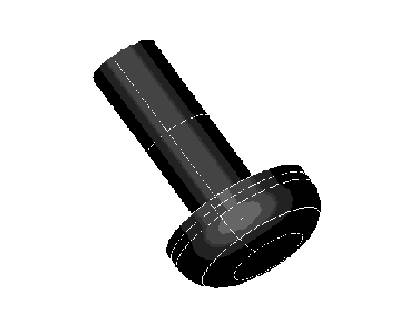A simple screw shape seen with shading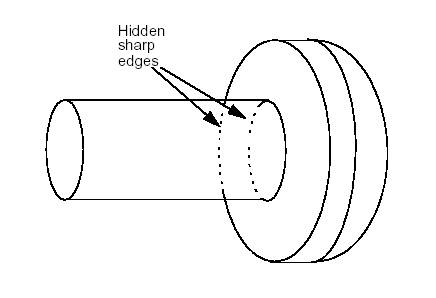An extraction showing hidden sharp edges

The following services are related to Hidden Lines Removal :

To pass a TopoDS_Shape to an HLRBRep_Algo object, use HLRBRep_Algo::Add. With an HLRBRep_PolyAlgo object, use HLRBRep_PolyAlgo::Load. If you wish to add several shapes, use Add or Load as often as necessary.

### Setting view parameters

HLRBRep_Algo::Projector and HLRBRep_PolyAlgo::Projector set a projector object which defines the parameters of the view. This object is an HLRAlgo_Projector.

### Computing the projections

HLRBRep_PolyAlgo::Update launches the calculation of outlines of the shape visualized by the HLRBRep_PolyAlgo framework.

In the case of HLRBRep_Algo, use HLRBRep_Algo::Update. With this algorithm, you must also call the method HLRBRep_Algo::Hide to calculate visible and hidden lines of the shape to be visualized. With an HLRBRep_PolyAlgo object, visible and hidden lines are computed by HLRBRep_PolyHLRToShape.

### Extracting edges

The classes HLRBRep_HLRToShape and HLRBRep_PolyHLRToShape present a range of extraction filters for an HLRBRep_Algo object and an HLRBRep_PolyAlgo object, respectively. They highlight the type of edge from the results calculated by the algorithm on a shape. With both extraction classes, you can highlight the following types of output:

• visible/hidden sharp edges;
• visible/hidden smooth edges;
• visible/hidden sewn edges;
• visible/hidden outline edges.

To perform extraction on an HLRBRep_PolyHLRToShape object, use HLRBRep_PolyHLRToShape::Update function.

For an HLRBRep_HLRToShape object built from an HLRBRepAlgo object you can also highlight:

• visible isoparameters and
• hidden isoparameters.

## Examples

### HLRBRep_Algo

// Build The algorithm object
myAlgo = new HLRBRep_Algo();
// Add Shapes into the algorithm
TopTools_ListIteratorOfListOfShape anIterator(myListOfShape);
for (;anIterator.More();anIterator.Next())
// Set The Projector (myProjector is a
HLRAlgo_Projector)
myAlgo-Projector(myProjector);
// Build HLR
myAlgo->Update();
// Set The Edge Status
myAlgo->Hide();
// Build the extraction object :
HLRBRep_HLRToShape aHLRToShape(myAlgo);
// extract the results :
TopoDS_Shape VCompound = aHLRToShape.VCompound();
TopoDS_Shape Rg1LineVCompound =
aHLRToShape.Rg1LineVCompound();
TopoDS_Shape RgNLineVCompound =
aHLRToShape.RgNLineVCompound();
TopoDS_Shape OutLineVCompound =
aHLRToShape.OutLineVCompound();
TopoDS_Shape IsoLineVCompound =
aHLRToShape.IsoLineVCompound();
TopoDS_Shape HCompound = aHLRToShape.HCompound();
TopoDS_Shape Rg1LineHCompound =
aHLRToShape.Rg1LineHCompound();
TopoDS_Shape RgNLineHCompound =
aHLRToShape.RgNLineHCompound();
TopoDS_Shape OutLineHCompound =
aHLRToShape.OutLineHCompound();
TopoDS_Shape IsoLineHCompound =
aHLRToShape.IsoLineHCompound();

### HLRBRep_PolyAlgo

// Build The algorithm object
myPolyAlgo = new HLRBRep_PolyAlgo();
// Add Shapes into the algorithm
TopTools_ListIteratorOfListOfShape
anIterator(myListOfShape);
for (;anIterator.More();anIterator.Next())
// Set The Projector (myProjector is a
HLRAlgo_Projector)
myPolyAlgo->Projector(myProjector);
// Build HLR
myPolyAlgo->Update();
// Build the extraction object :
HLRBRep_PolyHLRToShape aPolyHLRToShape;
aPolyHLRToShape.Update(myPolyAlgo);
// extract the results :
TopoDS_Shape VCompound =
aPolyHLRToShape.VCompound();
TopoDS_Shape Rg1LineVCompound =
aPolyHLRToShape.Rg1LineVCompound();
TopoDS_Shape RgNLineVCompound =
aPolyHLRToShape.RgNLineVCompound();
TopoDS_Shape OutLineVCompound =
aPolyHLRToShape.OutLineVCompound();
TopoDS_Shape HCompound =
aPolyHLRToShape.HCompound();
TopoDS_Shape Rg1LineHCompound =
aPolyHLRToShape.Rg1LineHCompound();
TopoDS_Shape RgNLineHCompound =
aPolyHLRToShape.RgNLineHCompound();
TopoDS_Shape OutLineHCompound =
aPolyHLRToShape.OutLineHCompound();

# Meshing

## Mesh presentations

In addition to support of exact geometrical representation of 3D objects Open CASCADE Technology provides functionality to work with tessellated representations of objects in form of meshes.

Open CASCADE Technology mesh functionality provides:

• data structures to store surface mesh data associated to shapes, and some basic algorithms to handle these data
• data structures and algorithms to build surface triangular mesh from BRep objects (shapes).
• tools to extend 3D visualization capabilities of Open CASCADE Technology with displaying meshes along with associated pre- and post-processor data.

Open CASCADE Technology includes two mesh converters:

• VRML converter translates Open CASCADE shapes to VRML 1.0 files (Virtual Reality Modeling Language). Open CASCADE shapes may be translated in two representations: shaded or wireframe. A shaded representation present shapes as sets of triangles computed by a mesh algorithm while a wireframe representation present shapes as sets of curves.
• STL converter translates Open CASCADE shapes to STL files. STL (STtereoLithography) format is widely used for rapid prototyping.

Besides, we can efficiently help you in the fields of surface and volume meshing algorithms, mesh optimization algorithms etc. If you require a qualified advice about meshing algorithms, do not hesitate to benefit from the expertise of our team in that domain.

The projects dealing with numerical simulation can benefit from using SALOME - an Open Source Framework for CAE with CAD data interfaces, generic Pre- and Post- F.E. processors and API for integrating F.E. solvers.

## Meshing algorithm

The algorithm of shape triangulation is provided by the functionality of BRepMesh_IncrementalMesh class, which adds a triangulation of the shape to its topological data structure. This triangulation is used to visualize the shape in shaded mode.

const Standard_Real aHeight = 25.0;
TopoDS_Shape aShape = aCylinder.Shape();
const Standard_Real aLinearDeflection = 0.01;
const Standard_Real anAngularDeflection = 0.5;
BRepMesh_IncrementalMesh aMesh(aShape, aLinearDeflection, Standard_False, anAngularDeflection);

The default meshing algorithm BRepMesh_IncrementalMesh has two major options to define triangulation – linear and angular deflections.

At the first step all edges from a face are discretized according to the specified parameters.

At the second step, the faces are tessellated. Linear deflection limits the distance between a curve and its tessellation, whereas angular deflection limits the angle between subsequent segments in a polyline.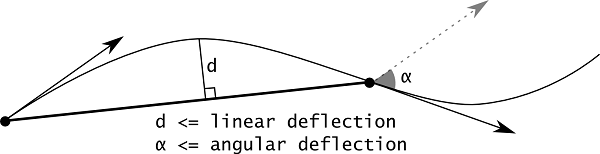Deflection parameters of BRepMesh_IncrementalMesh algorithm

Linear deflection limits the distance between triangles and the face interior.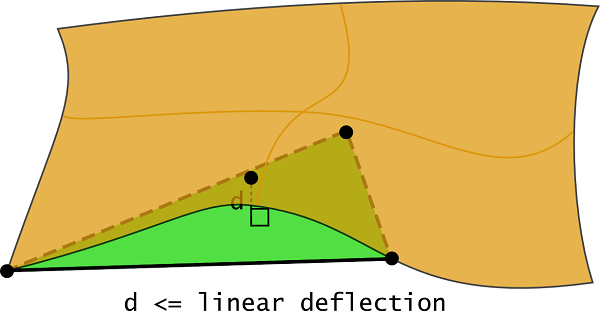Linear deflection

Note that if a given value of linear deflection is less than shape tolerance then the algorithm will skip this value and will take into account the shape tolerance.

The application should provide deflection parameters to compute a satisfactory mesh. Angular deflection is relatively simple and allows using a default value (12-20 degrees). Linear deflection has an absolute meaning and the application should provide the correct value for its models. Giving small values may result in a too huge mesh (consuming a lot of memory, which results in a long computation time and slow rendering) while big values result in an ugly mesh.

For an application working in dimensions known in advance it can be reasonable to use the absolute linear deflection for all models. This provides meshes according to metrics and precision used in the application (for example, it it is known that the model will be stored in meters, 0.004 m is enough for most tasks).

However, an application that imports models created in other applications may not use the same deflection for all models. Note that actually this is an abnormal situation and this application is probably just a viewer for CAD models with dimensions varying by an order of magnitude. This problem can be solved by introducing the concept of a relative linear deflection with some LOD (level of detail). The level of detail is a scale factor for absolute deflection, which is applied to model dimensions.

Meshing covers a shape with a triangular mesh. Other than hidden line removal, you can use meshing to transfer the shape to another tool: a manufacturing tool, a shading algorithm, a finite element algorithm, or a collision algorithm.

You can obtain information on the shape by first exploring it. To access triangulation of a face in the shape later, use BRepTool::Triangulation. To access a polygon, which is the approximation of an edge of the face, use BRepTool::PolygonOnTriangulation.

Generated on Wed Aug 30 2017 17:04:21 for Open CASCADE Technology by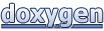1.8.13